# Creative Scala

March 2020# Foreword

Creative Scala is aimed at developers who have no prior experience in Scala. It is designed to give you a fun introduction to functional programming. We assume you have some very basic familiarity with another programming language but little or no experience with Scala or other functional languages.

We have three goals with this book:

1. To give an introduction to functional programming so that you can calculate and reason about programs, and pick up and understand other introductory books on functional programming.

2. To teach you enough Scala that you can explore your own interests in and using Scala.

3. To present all this in a fun, gentle, and interesting way via two-dimensional computer graphics.

Our motivation comes from our own experience learning programming, studying functional programming, and teaching Scala to commercial developers.

Firstly, we believe that functional programming is the future. Since we’re assuming you have little programming experience we won’t go into the details of the differences between functional programming and object-oriented programming that you may have already experienced. Suffice to say there are different ways to think about and write computer programs, and we’ve chosen the functional programming approach.

The reason for choosing functional programming are more interesting. It’s common to teach programming by what we call the “bag of syntax” approach. In this approach a programming language is taught a collection of syntactical features (variables, for loops, while loops, methods) and students are left to figure out on their own when to use each feature. We’ve seen this method fail both when we were undergraduates learning programming, and as postgraduates teaching programming, as students simply have no systematic way to break down a problem and turn it into code. The result is that many students dropped out due to the poor quality of teaching. The students that remained tended to, like us, already have extensive programming experience.

Let’s think back to primary school maths, specifically column addition. This is the basic way we’re taught to add up numbers when they’re too big to do in our head. So, for example, adding up 266 + 385, we would line up the columns, carry the tens and so on. Now maybe maths wasn’t your favorite subject but there are some important lessons here. The first is that we’re given a systematic way to arrive at the solution. We can calculate the solution once we realise this is a problem that requires column addition. The second point is that we don’t even have to understand why column addition works (though it helps) to use it. So long as we follow the steps we’ll get the correct answer.

The remarkable thing about functional programming is that it works like column addition. We have recipes that are guaranteed to give us the correct answer if we follow them correctly. We call this calculating a program. This is not to say that programming is without creativity, but the challenge is to understand the structure of the problem and once we’ve done that the recipe we should use follows immediately. The code itself is not the interesting part.

We’re teaching functional programming using Scala, but not Scala itself. Scala is a language that is in demand right now. Scala programmers can relatively easily get jobs in a variety of industries, and this is an important motivation for learning Scala. One of the reasons for Scala’s popularity is that is straddles object-oriented programming, the old way of programming, and functional programming. There is a lot of code written in an object-oriented style, and a lot of programmers who are used to that style. Scala gives a gentle way from object-oriented programming to functional programming. However this means Scala is a large language, and the interaction between the object-oriented and functional parts can be confusing. We believe that functional programming is much more effective than object-oriented programming, and for new programmers there is no need to add the confusion of learning object-oriented techniques at the same time. That can come later. Therefore this book is exclusively using the functional programming parts of Scala.

Finally, we’ve chosen what we hope is a fun method to explore functional programming and Scala: computer graphics. There are many introductions to Scala, but the majority use examples that either relate to business or mathematics. For example, one of the first exercises in the very popular Coursera course is to implement sets via indicator functions. We feel if you’re the type of person who likes directly working with these sort of concepts you already have plenty of content available. We want to target a different group: people who perhaps thought that maths was not for them but nonetheless have an interest or appreciation in the visual arts. We won’t lie: there is maths in this book, but we hope we manage to motivate and indeed visualise the concepts in a way that makes them less intimidating.

Although this book will give you the basic mental model required to become competent with Scala, you won’t finish knowing everything you need to be self-sufficient. For further advancement we recommend considering one of the many excellent Scala textbooks out there, including our own Essential Scala.

If you are working through the exercises on your own, we highly recommend joining our Gitter chat room to provide get help with the exercises and provide feedback on the book.

The text of Creative Scala is open source, as is the source code for the Doodle drawing library used in the exercises. You can grab the code from our GitHub account. Contact us on Gitter or by email if you would like to contribute.

Thanks for downloading and happy creative programming!

—Dave and Noel

## Notes on the Early Access Edition

This is an early access release of Creative Scala. There may be typos and other errors in the text and examples.

If you spot any mistakes or would like to provide feedback, please let us know via our Gitter chat room or by email:

## Acknowledgements

Creative Scala was written by Dave Gurnell and Noel Welsh. Many thanks to Richard Dallaway, Jonathan Ferguson, and the team at Underscore for their invaluable contributions and extensive proof reading.

Thanks also to the many people who pointed out errors or made suggestions to improve the book: Neil Moore; Kelley Robinson, Julie Pitt, and the other ScalaBridge organizers; d43; Matt Kohl; Alexa Kovachevich and all the other students who worked through Creative Scala at ScalaBridge, at another event, or on their own; and the many awesome members of the Scala community who gave us comments and suggestions in person. Finally, we have large amount of gratitude for Bridgewater, and particularly Lauren Cipicchio, who perhaps unknowingly funded a good portion of the initial development of the second version of the Creative Scala, and provided the first few rounds of students.

Finally, Creative Scala owes a large intellectual debt to the work of many researchers working in programming language theory and Computer Science education. In particular we’d like to highlight:

# 1 Getting Started

Our first step is to install the software we need to work with Creative Scala. We describe two pathways here:

1. Working with a text editor and a terminal. We recommend this setup to people completely new to programming as there are fewer moving parts.
2. Working with IntelliJ IDEA. We recommend this setup to people who are used to using an IDE or are uncomfortable with the terminal.

If you’re an experienced developer with a setup you are happy with just keep the tools you know and adapt the instructions below as needed.

If all this stuff is new to you, the rest of the chapter has some background.

## 1.1 Installing Terminal Software and a Text Editors

This section is our recommended setup for people new to programming, and describes how to setup Creative Scala with the terminal and a text editor. We need to install:

• the JVM;
• Git;
• a text editor; and
• the template project for Creative Scala.

### 1.1.1 OS X

Open the terminal. (Click the magnifying glass icon on the top righthand side of the toolbar. Type in “terminal”.)

Install Java. Type into the terminal

``java``

If this runs you already have Java installed. Otherwise it will prompt you to install Java.

Install homebrew. Paste into the terminal

``/usr/bin/ruby -e "\$(curl -fsSL https://raw.githubusercontent.com/Homebrew/install/master/install)"``

Install `git` using homebrew. At the terminal, type

``brew install git``

Now install the text editor Atom. Again type at the terminal

``brew install Caskroom/cask/atom``

Install Scala support inside Atom: Settings > Install > language-scala

Now we will use Git to get an SBT project that will work with Creative Scala. Type

``git clone https://github.com/underscoreio/creative-scala-template.git``

#### Sharing Your Work

There is an alternative setup that involves first forking the Creative Scala template project, and then cloning it to your computer. This is the setup to choose if you want to share your work with other people; for example you might be taking Creative Scala with a remote instructor or you might just (quite rightfully) be proud of your work.

In this setup you first fork the Creative Scala template. Then you make a clone of your fork. This alternative setup is described in more detail in the section on GitHub later in this chapter.

Now change to the directory we just created and run SBT.

``````cd creative-scala-template
./sbt.sh``````

SBT should start. Within SBT type `console`. Finally type

``Example.image.draw()``

and an image of three circles should appear!

If you’ve made it this far you’ve successfully installed all the software you need for work through Creative Scala.

The final step is to load Atom and use it to open `Example.scala`, which you can find in `src/main/scala`.

### 1.1.2 Windows

Download and install Java. Search for the “JDK” (Java development kit). This will take you to Oracle’s site. Accept their license and download the JDK. Run the installer you just downloaded.

Download and install Atom. Go to `https://atom.io/` and download Atom for Windows. Run the installer you’ve just downloaded.

Download and install Git. Go to `https://git-scm.com/` and download Git for Windows. Run the installer you’ve just downloaded. At the very end it gives you the option to open Git. Select that option. A window will open up with a command prompt. Type

``git clone https://github.com/underscoreio/creative-scala-template.git``

#### Sharing Your Work

There is an alternative setup that involves first forking the Creative Scala template project, and then cloning it to your computer. This is the setup to choose if you want to share your work with other people; for example you might be taking Creative Scala with a remote instructor or you might just (quite rightfully) be proud of your work.

In this setup you first fork the Creative Scala template. Then you make a clone of your fork. This alternative setup is described in more detail in the section on GitHub later in this chapter.

Open a normal command-prompt. Click on the Windows icon on the bottom left of the screen. In the search box enter “cmd” and run the program it finds. In the window that is opened up type

``cd creative-scala-template``

which will change into the directory of the Creative Scala template project we just downloaded. Type

``sbt.bat``

to start SBT. Within SBT type `console`. Finally type

``Example.image.draw()``

and an image of three circles should appear!

If you’ve made it this far you’ve successfully installed all the software you need for work through Creative Scala.

The final step is to load Atom and use it to open `Example.scala`, which you can find in the directory `src\main\scala`.

### 1.1.3 Linux

Follow the OS X instructions, using your distributions package manager to install software in place of Homebrew.

## 1.2 IntelliJ

IntelliJ is an integrated development environment (IDE) for Scala and other programming languages. It integrates an number of programming tools into one application, and we recommend it for people who are used to using other IDEs such as Visual Studiol or Eclipse.

Start by downloading and installing IntelliJ. You can use the free community edition for Creative Scala. When installing IntelliJ you will be asked a lot of questions. You can accept the defaults for the most part. When you are asked about “featured plugins”, make sure you install the Scala plug-in.

Once you have completed the configuration you will be presented with a dialog asking if you want to create a new project, import a project, open a file, or check out from version control. Choose “checkout from version control”, and select GitHub.

In the dialog box that opens change “Auth type” to Token. Now visit GitHub in a web browser. Select your account (top right hand of the page). Choose “Settings” and then choose “Personal access tokens”. Generate a token. Call it “intellij” and select the “repo” check box. Copy the long string of numbers and text and paste it into the “Token” box. Now login to GitHub.

Install the SBT console add-on.

## 1.3 Background

This section gives some background information on some of the tools we’ll be using. If you’re an experienced developer a lot of this will be old hat, and you can skip it. If you’re not, this will hopefully give some useful context to the software we’ll be working with.

### 1.3.1 The Terminal

Back when the world was young and computing was in its infancy, the common user interface of graphical windows, a cursor controlled by a mouse, and interaction by direct manipulation didn’t exist. Instead users typed in commands at a device called a terminal. The direct manipulation interface is superior for most uses, but there are some cases for which the terminal or command line is preferable. For example, if we wanted to work out how much space was used by all the files which names starting with `data` in Linux or OS X we can execute the command

``du -hs data*``

We can break this down into three components:

• the command `du` means disk usage;
• the flags `-hs` mean to print a human readable summary; and
• the pattern `data*` means all the files whose names begin with `data`.

Doing this with a direct manipulation interface would be much more time consuming.

The command line has a steep learning curve, but the reward is an extremely powerful tool. Our usage of the terminal will be very limited, so don’t worry if you find the example above intimidating!

### 1.3.2 Text Editors

You’re probably used to writing documents in a word processor. A word processor allows us to write text and control the formatting of how it appears on the (increasingly rare) printed page. A word processor includes powerful commands, such as a spell checker and automatic table of contents generation, to make editing prose easier.

A text editor is like a word processor for code. Whereas a word processor is concerned about visual presentation of text, a text editor has many programming specific functions. Typical examples include powerful tools to search and replace text, and the ability to quickly jump between the many different files that make up a project.

Text editors date back to the days of terminals and perhaps surprisingly some of these tools are still in use. The two main ancient and glorious text editors that survive are called Emacs and Vim. They have very different approaches (except when they don’t) and developers tend to use one or the other. I’ve been using Emacs for about twenty years, and thus I know in my bones that Emacs is the greatest of all possible text editors and Vim users are knuckle-draggers lumbered with poor taste and an inferior tool. Vim users no doubt think the same about me.

If there is one thing that unites Vim and Emacs users it’s the sure knowledge that new-fangled text editors like Sublime Text and Atom are bringing about the downfall of our civilization. Nonetheless we recommend using Atom if you’re new to this text editing game. Both Vim and Emacs were created before the common interfaces in use today were created, and using them requires learning a very different way of working.

### 1.3.3 The Compiler

The code we write in a text editor is not in a form that a computer can run. A compiler translates it into something the computer can run. As it does this it performs certain checks on the code. If these checks don’t pass the code won’t be compiled and the compiler will print an error message instead. We’ll learn more about what the compiler can check and what it can’t in the rest of this book.

When we said the compiler translates the code is something the computer can run, this is not the complete truth in the case of Scala. The output of the compiler is something called bytecode, and another program, called the Java Virtual Machine (JVM), runs this code1.

### 1.3.4 Integrated Development Environments

Integrated development environments (IDEs) are an alternative approach that combine a text editor, a compiler, and several other programmer tools into a single program. Some people swear by IDEs, while some people prefer to use the terminal and a text editor. Our recommendation if you’re new to programming is to take the terminal-and-text-editor approach. If you’re already used to an IDE then IntelliJ IDEA is currently the best IDE for Scala development.

### 1.3.5 Version Control

Version control is the final tool we’ll use. A version control system is a program that allows us to keep a record of all the changes that have been made to a group of files. It’s very useful for allowing multiple people to work on a project at the same time, and it ensures people don’t accidentally overwrite each others changes. This is not a huge concern in Creative Scala, but it is good to get some exposure to version control now.

The version control software we’ll use is called Git. It’s powerful but complex. The good news is we don’t need to learn much about Git. Most of our use of Git will be via a website called GitHub, which allows people to share software that is stored in Git. We use GitHub to share the software used in Creative Scala.

### 1.3.6 Onward!

Now that we’ve got some background, let’s move on to installing the software we need to write Scala code.

## 1.4 GitHub

We have created a template for you that will get you set up with all the code you need to work through Creative Scala. This template is stored on GitHub, a website for sharing code.

You can copy the template onto your computer, which Git calls cloning, but this means you won’t be able to save any changes you make back to GitHub where other people can view them.

If you want to be able to share your changes, you need to make a copy of the template project on GitHub that you own. Git calls this forking. You fork the repository on GitHub and then clone your fork to your computer. Then you can save your changes back to your fork on GitHub.

To start this process you need to create a GitHub account, if you do not have one already.

Once you have an account, visit the template project in your browser. At the top right is button called “Fork”. Press this button to create your own copy of the template. You will be taken to a web page displaying your own fork of the template. Remember the name of this repository. It should be something like `yourname/creative-scala-template` where `yourname` is your GitHub user name.

Now cloning your fork is as simple as running this command and replacing `yourname` with your actual GitHub user name.

``git clone git@github.com:yourname/creative-scala-template.git``

Now any changes you make can be sent back to your fork on GitHub. The process for doing this in Git is a bit involved. When you’ve made a change you must:

• `add` the change to what’s called Git’s index;
• `commit` the change; and finally
• `push` the change to the fork.

Here’s an example of using the command line to do this.

``````git add
git commit -m "Explain here what you did"
git push``````

GitHub makes a nice free graphical tool for using Git, called GitHub Desktop. It’s probably the easiest way to use Git when you’re getting started.

# 2 Expressions, Values, and Types

Scala programs have three fundamental building blocks: expressions, values, and types. In this section, we explore these concepts.

Here’s a very simple expression:

``1 + 2``

An expression is a fragment of Scala code. We can write expressions in a text editor, or on a piece of paper, or on a wall; expressions are like writing. Just like writing must be read for it to have any effect on the world (and the reader has to understand the language the writing is written in), the computer must run an expression for it to have an effect. The result of running an expression is a value. Values live in the computer’s memory, in the same way that the result of reading some writing lives in the reader’s head. We will also say expressions are evaluated or executed to describe the process of transforming them into values.

We can evaluate expressions immediately by writing them at the console and pressing “Enter” (or “Return”). Try it now.

``````1 + 2
// res1: Int = 3``````

The console responds with the value the expression evaluates to, and the type of the expression.

The expression `1 + 2` evaluates to the value `3`. We can write down the number three here on the page, but the real value is something stored in the computer’s memory. In this case, it is a 32-bit integer represented in two’s-complement. The meaning of “32-bit integer represented in two’s-complement” is not important. We just mention it to emphasize the fact the computer’s representation of the value `3` is the true value, not the numeral written here or displayed by the console.

Types are the final piece of the puzzle. A type describes a set of values. The expression `1 + 2` has the type `Int`, which means the value the expression evaluates to will be one of the over four billion values the computer understands to be integers. We determine the type of an expression without running it, which is why the type `Int` doesn’t tell us which specific value the expression evalutes to.

Before a Scala program is run, it must be compiled. Compilation checks that a program makes sense. It must be syntactically correct, meaning it must be written according to the rules of Scala. For example `(1 + 2)` is syntactically correct, but `(1 + 2` is not because there is no `)` to match the `(`. It must also type check, meaning the types must be correct for the operations we’re trying to do. `1 + 2` type checks (we are adding integers), but `1.toUpperCase` does not (there is no concept of upper and lower case for integers.)

Only programs that successfully compile can be run. We can think of compilation as being analogous to the rules of grammar in writing. The sentence “FaRf fjrmn;l df.fd” is syntactically incorrect in English. The arrangement of letters doesn’t form any words. The sentence “dog fly a here no” is made out of valid words but their arrangement breaks the rules of grammar—analogous to the type checks that Scala performs.

It is important to remember that type checking is done before a program runs. If you have used a language like Python or Javascript, which are sometimes called “dynamically typed”, there is no type checking done before a program runs. In a “statically typed” language like Scala the type checking catches some potential errors for us before we run the code. What is sometimes called a type in a dynamically typed language is not a type as we understand it. Types, for us, exist at at the time when a program is compiled, which we will call compile time. At time when a program runs, which we call run time, we have only values. Values may record some information about the type of the expression that created them. If they do we call these tags, or sometimes boxes. Not all values are tagged or boxed. Avoiding tagging, which is also called type erasure, allows for more efficient programs.

## 2.1 Literal Expressions

We’ll now start to explore the various forms of expressions in Scala, starting with the simplest expressions, literals. Here’s a literal expression:

``````3
// res0: Int = 3``````

A literal evaluates to “itself.” How we write the expression and how the console prints the value are the same. Remember though, there is a difference between the written representation of a value and its actual representation in the computer’s memory.

Scala has many different forms of literals. We’ve already seen `Int` literals. There is a different type, and a different literal syntax, for what are called floating point numbers. This corresponds to a computer’s approximation of the real numbers. Here’s an example:

``````0.1
// res1: Double = 0.1``````

As you can see, the type is called `Double`.

Numbers are well and good, but what about text? Scala’s `String` type represents a sequence of characters. We write literal strings by putting their contents in double quotes.

``````"To be fond of dancing was a certain step towards falling in love."
// res2: String = "To be fond of dancing was a certain step towards falling in love."``````

Sometimes we want to write strings that span several lines. We can do this by using triple double quotes, as below.

``````"""
A new, a vast, and a powerful language is developed for the future use of analysis,
in which to wield its truths so that these may become of more speedy and accurate
practical application for the purposes of mankind than the means hitherto in our
possession have rendered possible.

-- Ada Lovelace, the world's first programmer
"""
// res3: String = """
// A new, a vast, and a powerful language is developed for the future use of analysis,
// in which to wield its truths so that these may become of more speedy and accurate
// practical application for the purposes of mankind than the means hitherto in our
// possession have rendered possible.
//
//   -- Ada Lovelace, the world's first programmer
// """``````

A `String` is a sequence of characters. Characters themselves have a type, `Char`, and character literals are written in single quotes.

``````'a'
// res4: Char = 'a'``````

Finally we’ll look at the literal representations of the `Boolean` type, named after English logician George Boole. This fancy name just means a value that can be either `true` or `false`, and this indeed is how we write boolean literals.

``````true
// res5: Boolean = true
false
// res6: Boolean = false``````

With literal expressions, we can create values, but we won’t get very far if we can’t somehow manipulate the values we’ve created. We’ve seen a few examples of more complex expressions like `1 + 2`. In the next section, we’ll learn about objects and methods, which will allow us to understand how this, and more interesting expressions, work.

## 2.2 Values are Objects

In Scala all values are objects. An object is a grouping of data and operations on that data. For example, 2 is an object. The data is the integer 2, and the operations on that data are familiar operations like +, -, and so on. We call operations of an object the object’s methods.

### 2.2.1 Method Calls

We interact with objects by calling or invoking methods. For example, we can get the uppercase version of a `String` by calling its `toUpperCase` method.

``````"Titan!".toUpperCase
// res0: String = "TITAN!"``````

Some methods accept parameters or arguments, which control how the method works. The `take` method, for example, takes characters from a `String`. We must pass a parameter to `take` to specify how many characters we want.

``````"Gilgamesh went abroad in the world".take(3)
// res1: String = "Gil"
"Gilgamesh went abroad in the world".take(9)
// res2: String = "Gilgamesh"``````

A method call is an expression, and thus evaluates to an object. This means we can chain method calls together to make more complex programs:

``````"Titan!".toUpperCase.toLowerCase
// res3: String = "titan!"``````

#### Method Call Syntax

The syntax for a method call is

``anExpression.methodName(param1, ...)``

or

``anExpression.methodName``

where

• `anExpression` is any expression (which evaluates to an object)
• `methodName` is the name of the method
• the optional `param1, ...` are one or more expressions evaluating to the parameters to the method.

### 2.2.2 Operators

We have said that all values are objects, and we call methods with the syntax `object.methodName(parameter)`. How then do we explain expressions like `1 + 2`?

In Scala, and expression written `a.b(c)` can be written `a b c`. So these are equivalent:

``````1 + 2
// res4: Int = 3
1.+(2)
// res5: Int = 3``````

This first way of calling a method is known an operator style.

#### Infix Operator Notation

Any Scala expression written `a.b(c)` can also be written `a b c`.

Note that `a b c d e` is equivalent to `a.b(c).d(e)`, not `a.b(c, d, e)`.

## 2.3 Types

Now that we can write more complex expressions, we can talk a little more about types.

One use of types is stopping us from calling methods that don’t exist. The type of an expression tells the compiler what methods exist on the value it evaluates to. Our code won’t compile if we try to call to a method that doesn’t exist. Here are some simple examples.

``````"Brontë" / "Austen"
1.take(2)
// error: value / is not a member of String
// "Brontë" / "Austen"
// ^^^^^^^^^^
// error: value take is not a member of Int
// 1.take(2)
// ^^^^^^``````

It really is the type of the expression that determines what methods we can call, which we can demonstrate by calling methods on the result of more complex expressions.

``````(1 + 3).take(1)
// error: value take is not a member of Int
// (1 + 3).take(1)
//  ^^^^^^^^^^^``````

This process of type checking also applies to the parameter of methods.

``````1.min("zero")
// error: type mismatch;
//  found   : String("zero")
//  required: Int
// 1.min("zero")
//       ^^^^^^``````

Types are a property of expressions and thus exist at compile time (as we have discussed before.) This means we can determine the type of an expression even if evaluating it results in an error at run time. For example, dividing an `Int` by zero causes a run-time error.

``````1 / 0
// java.lang.ArithmeticException: / by zero
//  at repl.Session\$App\$\$anonfun\$4.apply\$mcI\$sp(types.md:27)
//  at repl.Session\$App\$\$anonfun\$4.apply(types.md:27)
//  at repl.Session\$App\$\$anonfun\$4.apply(types.md:27)``````

The expression `1 / 0` still has a type, and we can get that type from the console as shown below.

``````:type 1 / 0
// Int``````

We can also write a compound expression including a sub-expression that will fail at run-time.

``````(2 + (1 / 0) + 3)
// java.lang.ArithmeticException: / by zero
//  at repl.Session\$App\$\$anonfun\$5.apply\$mcI\$sp(types.md:35)
//  at repl.Session\$App\$\$anonfun\$5.apply(types.md:35)
//  at repl.Session\$App\$\$anonfun\$5.apply(types.md:35)``````

This expression also has a type.

``````:type (2 + (1 / 0) + 3)
// Int``````

## 2.4 Exercises

#### 2.4.0.1 Arithmetic

Write an expression using integer literals, addition, and subtraction that evaluates to 42.

This exercise is just about getting used to writing Scala code. Here is one possible solution.

``````1 + 43 - 2
// res0: Int = 42``````

#### 2.4.0.2 Appending Strings

Join together two strings (known as appending strings) using the `++` method. Write equivalent expressions using both the normal method call style and the operator style.

Something like the below should do.

``````"It is a truth ".++("universally acknowledged")
// res1: String = "It is a truth universally acknowledged"
"It is a truth " ++ "universally acknowledged"
// res2: String = "It is a truth universally acknowledged"``````

#### 2.4.0.3 Precedence

In mathematics we learned that some operators take precedence over others. For example, in the expression `1 + 2 * 3` we should do the multiplication before the addition. Do the same rules hold in Scala?

A bit of exploration at the console should convince you that yes, Scala does maintain the standard precedence rules. The example below demonstrates this.

``````1 + 2 * 3
// res3: Int = 7
1 + (2 * 3)
// res4: Int = 7
(1 + 2) * 3
// res5: Int = 9``````

#### 2.4.0.4 Types and Values

Which of the following expressions will not compile? Of the expressions that will compile, what is their type? Which expressions fail at run-time?

``````1 + 2

"3".toInt

"Electric blue".toInt
// java.lang.NumberFormatException: For input string: "Electric blue"
//  at java.lang.NumberFormatException.forInputString(NumberFormatException.java:65)
//  at java.lang.Integer.parseInt(Integer.java:580)
//  at java.lang.Integer.parseInt(Integer.java:615)
//  at scala.collection.immutable.StringLike.toInt(StringLike.scala:304)
//  at scala.collection.immutable.StringLike.toInt\$(StringLike.scala:304)
//  at scala.collection.immutable.StringOps.toInt(StringOps.scala:33)
//  at repl.Session\$App\$\$anonfun\$9.apply\$mcI\$sp(exercises.md:48)
//  at repl.Session\$App\$\$anonfun\$9.apply(exercises.md:48)
//  at repl.Session\$App\$\$anonfun\$9.apply(exercises.md:48)

"Electric blue".take(1)

"Electric blue".take("blue")

1 + ("Moonage daydream".indexOf("N"))

1 / 1 + ("Moonage daydream".indexOf("N"))

1 / (1 + ("Moonage daydream".indexOf("N")))
// java.lang.ArithmeticException: / by zero
//  at repl.Session\$App\$\$anonfun\$14.apply\$mcI\$sp(exercises.md:80)
//  at repl.Session\$App\$\$anonfun\$14.apply(exercises.md:80)
//  at repl.Session\$App\$\$anonfun\$14.apply(exercises.md:80)``````
``````1 + 2
// res12: Int = 3``````

This expression has type `Int` and evaluates to `3`.

``````"3".toInt
// res13: Int = 3``````

This expression has type `Int` and evaluates to `3`.

``````"Electric blue".toInt
// java.lang.NumberFormatException: For input string: "Electric blue"
//  at java.lang.NumberFormatException.forInputString(NumberFormatException.java:65)
//  at java.lang.Integer.parseInt(Integer.java:580)
//  at java.lang.Integer.parseInt(Integer.java:615)
//  at scala.collection.immutable.StringLike.toInt(StringLike.scala:304)
//  at scala.collection.immutable.StringLike.toInt\$(StringLike.scala:304)
//  at scala.collection.immutable.StringOps.toInt(StringOps.scala:33)
//  at repl.Session\$App\$\$anonfun\$17.apply\$mcI\$sp(exercises.md:100)
//  at repl.Session\$App\$\$anonfun\$17.apply(exercises.md:100)
//  at repl.Session\$App\$\$anonfun\$17.apply(exercises.md:100)``````

This expression has type `Int` but fails at run-time.

``````"Electric blue".take(1)
// res14: String = "E"``````

This expression has type `String` and evaluates to `"E"`.

``````"Electric blue".take("blue")
// error: type mismatch;
//  found   : String("blue")
//  required: Int
// "Electric blue".take("blue")
//                      ^^^^^^``````

This expression fails at compile-time and hence has no type.

``````1 + ("Moonage daydream".indexOf("N"))
// res16: Int = 0``````

This expression has type `Int` and evaluates to `0`.

``````1 / 1 + ("Moonage daydream".indexOf("N"))
// res17: Int = 0``````

This expression has type `Int` and, due to precedence, evaluates to `(1 / 1) + -1`, which is `0`.

``````1 / (1 + ("Moonage daydream".indexOf("N")))
// java.lang.ArithmeticException: / by zero
//  at repl.Session\$App\$\$anonfun\$22.apply\$mcI\$sp(exercises.md:132)
//  at repl.Session\$App\$\$anonfun\$22.apply(exercises.md:132)
//  at repl.Session\$App\$\$anonfun\$22.apply(exercises.md:132)``````

This expression has type `Int` but fails at run-time with a division by zero.

#### 2.4.0.5 Floating Point Failings

When we introduced Doubles, I said they are an approximation to the real numbers. Why do you think this is? Think about representing numbers like ⅓ and π. How much space would it take to represent these numbers in decimal?

`Double` is an approximation because it has the fit within the computer’s finite memory. A `Double` takes up precisely 64-bits, which is enough space to store a lot of digits but not enough to store a number that, like π, has an infinite expansion.

The number ⅓ also has an infinite expansion in decimal. Because Doubles are stored in binary there are some numbers that can be represented in a finite number of decimal digits but have no finite representation in binary. 0.1 turns out to be one such number.

In general, floating point numbers can lead to nasty surprises if you expect them to act like the reals. They are fine for our purposes in Creative Scala, but don’t go using them to write accounting software!

#### 2.4.0.6 Beyond Expressions

In our current model of computation there are only three components: expressions (program text) with types, that evaluate to values (something within the computer’s memory). Is this sufficient? Could we write a stock market or a computer game with just this model? Can you think of ways to extend this model?

This is very open ended question. There are several ways to go beyond the model we have so far.

To be useful our programs must be capable of creating effects—changes in the world that go beyond the computer’s memory. For example, displaying things on the screen, making sound, sending messages to other computers, and the like. The console implicitly does some of this for us, by printing values on the screen. We’ll need to go a bit beyond that for more useful programs.

We also don’t have any way to define our own objects and methods, or reuse values in our programs. If we want to, say, use someone’s name across a program we have to repeat that name everywhere. We need more methods of abstraction and that’s what we’ll turn to soon.

# 3 Computing With Pictures

So far we have computed using numbers, strings, and other simple objects. From here on out we will focus our attention on computing with pictures, and later with animations. Pictures offer us more immediate creative opportunities, and they make our program output tangible in a way that other methods can’t deliver.

We’ll use a library called Doodle to help us with creating graphics. In this chapter we will learn the basics of Doodle.

If you run the examples from the SBT console within Doodle they will just work. If not, you will need to start your code with the following imports to make Doodle available.

``````import doodle.core._
import doodle.image._
import doodle.image.syntax._
import doodle.image.syntax.core._
import doodle.java2d._``````

## 3.1 Images

Let’s start with some simple shapes, programming at the console as we’ve done before.

``````Image.circle(10)
// res0: Image = Circle(10.0)``````

What is happening here? `Image` is an object and `circle` a method on that object. We pass to `circle` a parameter, `10` that gives the diameter of the circle we’re constructing. Note the type of the result—an `Image`.

``````Image.circle(10)
// res1: Image = Circle(10.0)``````

We draw the circle by calling the `draw` method.

``Image.circle(10).draw()``

A window should appear as shown in fig. 1.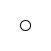Figure 1: A circle

Doodle supports a handful of “primitive” images: circles, rectangles, and triangles. Let’s try drawing a rectangle.

``Image.rectangle(100, 50).draw()``

The output is shown in fig. 2.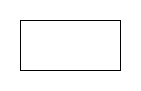Figure 2: A rectangle

Finally let’s try a triangle, for which the output is shown in fig. 3.

``Image.triangle(60, 40).draw()``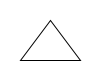Figure 3: A triangle

### Exercises

#### I Go Round in Circles

Create circles that are 1, 10, and 100 units wide. Now draw them!

In this exercise we’re checking that our Doodle install is working correctly and we’re getting used to using the library. One of the important points in Doodle is we separate defining the image from drawing the image. We’ll talk more about this throughout the book.

We can create circles with the code below.

``````Image.circle(1)
Image.circle(10)
Image.circle(100)``````

We can draw the circles by calling the `draw` method on each circle.

``````Image.circle(1).draw()
Image.circle(10).draw()
Image.circle(100).draw()``````

#### My Type of Art

What is the type of a circle? A rectangle? A triangle?

They all have type `Image`, as we can tell from the console.

``````:type Image.circle(10)
// doodle.core.Image
:type Image.rectangle(10, 10)
// doodle.core.Image
:type Image.triangle(10, 10)
// doodle.core.Image``````

#### Not My Type of Art

What’s the type of drawing an image? What does this mean?

Once again, we can ask the console this quesstion.

``````:type Image.circle(10).draw()
// Unit``````

We see that the type of drawing an image is `Unit`. `Unit` is the type of expressions that have no interesting value to return. This is the case for `draw`; we call it because we want something to appear on the screen, not because we have a use for the value it returns. There is only one value with type `Unit`. This value is also called unit, which written as a literal expression is `()`

You’ll note that the console doesn’t print unit by default.

``()``

We can ask the console for the type to show that there really is unit here.

``````:type ()
// Unit``````

## 3.2 Layout

We can seen how to create primitive images. We can combine together images using layouts methods to create more complex images. Try the following code—you should see a circle and a rectangle displayed beside one another, as in fig. 4.

``(Image.circle(10).beside(Image.rectangle(10, 20))).draw()``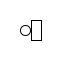Figure 4: A circle beside a rectangle

`Image` contains several layout methods for combining images, described in tbl. 1. Try them out now to see what they do.

Table 1: Layout methods available in Doodle
Method Parameter Description Example

`beside`

`Image`

Places images horizontally next to one another

`Image.circle(10) .beside(Image.circle(10))`

`above`

`Image`

Places images vertically next to one another

`Image.circle(10) .above(Image.circle(10))`

`below`

`Image`

Places images vertically next to one another

`Image.circle(10) .below(Image.circle(10))`

`on`

`Image`

Places images centered on top of one another

`Image.circle(10) .on(Image.circle(10))`

`under`

`Image`

Places images centered on top of one another

`Image.circle(10) .under(Image.circle(10))`

### Exercises

#### The Width of a Circle

Create the picture fig. 5 using the layout methods and basic images we’ve covered so far.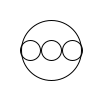Figure 5: The width of a circle

It’s three small circles on top of a bigger circle, and we can just about state this as is in code.

``````(Image
.circle(20)
.beside(Image.circle(20))
.beside(Image.circle(20))).on(Image.circle(60))
// res0: Image = On(
//   Beside(Beside(Circle(20.0), Circle(20.0)), Circle(20.0)),
//   Circle(60.0)
// )``````

## 3.3 Color

In addition to layout, Doodle has some simple operators to add a splash of colour to our images. Try these out the methods described in tbl. 2 to see how they work.

Table 2: Some of the methods to add color to images in Doodle.
Method Parameter Description Example

`fillColor`

`Color`

Fills the image with the specified color.

`Image.circle(10) .fillColor(Color.red)`

`strokeColor`

`Color`

Outlines the image with the specified color.

`Image.circle(10) .strokeColor(Color.blue)`

`strokeWdith`

`Double`

Sets the width of the image outline.

`Image.circle(10) .strokeWidth(3)`

`noFill`

None

Removes any fill from the image.

`Image.circle(10).noFill`

`noStroke`

None

Removes any stroke from the image.

`Image.circle(10).noStroke`

Doodle has various ways of creating colours. The simplest are the predefined colours in CommonColors.scala. Some of the most commonly used are described in tbl. 3.

Table 3: Some of the most common predefined colors.
Color Type Example

`Color.red`

`Color`

`Image.circle(10).fillColor(Color.red)`

`Color.blue`

`Color`

`Image.circle(10).fillColor(Color.blue)`

`Color.green`

`Color`

`Image.circle(10).fillColor(Color.green)`

`Color.black`

`Color`

`Image.circle(10).fillColor(Color.black)`

`Color.white`

`Color`

`Image.circle(10).fillColor(Color.white)`

`Color.gray`

`Color`

`Image.circle(10).fillColor(Color.gray)`

`Color.brown`

`Color`

`Image.circle(10).fillColor(Color.brown)`

### Exercises

#### Evil Eye

Make the image in fig. 6, designed to look like a traditional amulet protecting against the evil eye. I used `cornflowerBlue` for the iris, and `darkBlue` for the outer color, but experiment with your own choices!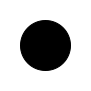Figure 6: No evil eyes here!

Here’s my amulet:

``````Image
.circle(10)
.fillColor(Color.black)
.on(Image.circle(20).fillColor(Color.cornflowerBlue))
.on(Image.circle(30).fillColor(Color.white))
.on(Image.circle(50).fillColor(Color.darkBlue))
// res0: Image = On(
//   On(
//     On(
//       FillColor(
//         Circle(10.0),
//         RGBA(
//           UnsignedByte(-128),
//           UnsignedByte(-128),
//           UnsignedByte(-128),
//           Normalized(1.0)
//         )
//       ),
//       FillColor(
//         Circle(20.0),
//         RGBA(
//           UnsignedByte(-28),
//           UnsignedByte(21),
//           UnsignedByte(109),
//           Normalized(1.0)
//         )
//       )
//     ),
//     FillColor(
//       Circle(30.0),
//       RGBA(
//         UnsignedByte(127),
//         UnsignedByte(127),
//         UnsignedByte(127),
//         Normalized(1.0)
//       )
//     )
//   ),
//   FillColor(
//     Circle(50.0),
//     RGBA(
//       UnsignedByte(-128),
//       UnsignedByte(-128),
//       UnsignedByte(11),
//       Normalized(1.0)
//     )
//   )
// )``````

## 3.4 Creating Colors

We’ve seen how to use predefined colors in our images. What about creating our own colors? In this section we will see how to create colors of our own, and transform existing colors into new ones.

### 3.4.1 RGB Colors

Computers work with colors defined by mixing together different amounts of red, green, and blue. This “RGB” model is an additive model of color, which means adding more colors gets us closer to white. This is the oppositive of paint, which is a subtractive model where adding more paints gets us closer to black. Each red, green, or blue component can have a value between zero and 255. If all three components are set to the maximum of 255 we get pure white. If all components are zero we get black.

We can create our own RGB colors using the `rgb` method on the `Color` object. This method takes three parameters: the red, green, and blue components. These are numbers between 0 and 255, called an `UnsignedByte`2. There is no literal expression for `UnsignedByte` like there is for `Int`, so we must convert an `Int` to `UnsignedByte`. We can do this with the `uByte` method. An `Int` can take on more values that an `UnsignedByte`, so if the number is too small or too large to be represented as a `UnsignedByte` it will be converted to the closest values is the range 0 to 255. These examples illustrate the conversion.

``````0.uByte.get
// res0: Int = 0
255.uByte.get
// res1: Int = 255
128.uByte.get
// res2: Int = 128
-100.uByte.get // Too small, is transformed to 0
// res3: Int = 0 // Too small, is transformed to 0
1000.uByte.get // Too big, is transformed to 255
// res4: Int = 255``````

(Note that `UnsignedByte` is a feature of Doodle. It is not something provided by Scala.)

Now we know how to construct `UnsignedBytes` we can make RGB colors.

``````Color.rgb(255.uByte, 255.uByte, 255.uByte) // White
Color.rgb(0.uByte, 0.uByte, 0.uByte) // Black
Color.rgb(255.uByte, 0.uByte, 0.uByte) // Red``````

### 3.4.2 HSL Colors

The RGB color representation is not very easy to use. The hue-saturation-lightness (HSL) format more closely corresponds to how we perceive color. In this representation a color consists of:

• hue, which is an angle between 0 and 360 degrees giving a rotation around the color wheel.
• saturation, which is a number between 0 and 1 giving the intensity of the color from a drab gray to a pure color; and
• lightness between 0 and 1 giving the color a brightness varying from black to pure white.

fig. 7 shows how colors vary as we change hue and lightness, and fig. 8 shows the effect of changing saturation.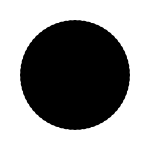Figure 7: A color wheel showing changes in hue (rotations) and lightness (distance from the center) with saturation fixed at 1.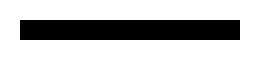Figure 8: A gradient showing how changing saturation effects color, with hue and lightness held constant. Saturation is zero on the left and one on the right.

We can construct a color in the HSL representation using the `Color.hsl` method. This method takes as parameters the hue, saturation, and lightness. The hue is an `Angle`. We can convert a `Double` to an `Angle` using the `degrees` (or `radians`) methods.

``````0.degrees
// res8: Angle = Angle(0.0)
180.degrees
// res9: Angle = Angle(3.141592653589793)
3.14.radians
// res10: Angle = Angle(3.14)``````

Saturation and lightness are both `Doubles` that should be between 0.0 and 1.0. Values outside this range will be converted to the closest number within the range.

We can now create colors using the HSL representation.

``Color.hsl(0.degrees, 0.8, 0.6) // A pastel red``

To view this color we can render it in a picture. See fig. 9 for an example.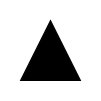Figure 9: Rendering pastel red in a triangle

### 3.4.3 Manipulating Colors

The effectiveness of a composition often depends as much on the relationships between colors as the actual colors used. Colors have several methods that allow us to create a new color from an existing one. The most commonly used ones are:

• `spin`, which rotates the hue by an `Angle`;
• `saturate` and `desaturate`, which respectively add and subtract a `Normalised` value from the color; and
• `lighten` and `darken`, which respectively add and subtract a `Normalised` value from the lightness.

For example,

``````Image.circle(100)
.fillColor(Color.red)
.beside(Image.circle(100).fillColor(Color.red.spin(15.degrees)))
.beside(Image.circle(100).fillColor(Color.red.spin(30.degrees)))
.strokeWidth(5.0)``````

produces fig. 10.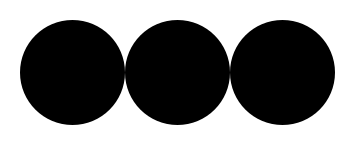Figure 10: Three circles, starting with Color.red and spinning by 15 degrees for each successive circle

Here’s a similar example, this time manipulating saturation and lightness, shown in fig. 11.

``````Image.circle(40)
.fillColor(Color.red.darken(0.2.normalized))
.beside(Image.circle(40).fillColor(Color.red))
.beside(Image.circle(40).fillColor((Color.red.lighten(0.2.normalized))))
.above(Image.rectangle(40, 40).fillColor(Color.red.desaturate(0.6.normalized))
.beside(Image.rectangle(40,40).fillColor(Color.red.desaturate(0.3.normalized)))
.beside(Image.rectangle(40,40).fillColor(Color.red)))``````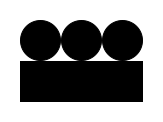Figure 11: The top three circles show the effect of changing lightness, and the bottom three squares show the effect of changing saturation.

### 3.4.4 Transparency

We can also add a degree of transparency to our colors, by adding an alpha value. An alpha value of 0.0 indicates a completely transparent color, while a color with an alpha of 1.0 is completely opaque. The methods `Color.rgba` and `Color.hsla` have a fourth parameter that is a `Normalized` alpha value. We can also create a new color with a different transparency by using the `alpha` method on a color. Here’s an example, shown in fig. 12.

``````Image.circle(40)
.fillColor(Color.red.alpha(0.5.normalized))
.beside(Image.circle(40).fillColor(Color.blue.alpha(0.5.normalized)))
.on(Image.circle(40).fillColor(Color.green.alpha(0.5.normalized)))``````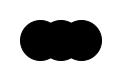Figure 12: Circles with alpha of 0.5 showing transparency

### Exercises

#### Analogous Triangles

Create three triangles, arranged in a triangle, with analogous colors. Analogous colors are colors that are similar in hue. See a (fairly elaborate) example in fig. 13.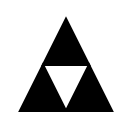Figure 13: Analogous triangles. The colors chosen are variations on `darkSlateBlue`

These sort of examples are getting a bit too long to write out at the console. We’ll look at a way around this next.

``````Image.triangle(40, 40)
.strokeWidth(6.0)
.strokeColor(Color.darkSlateBlue)
.fillColor(Color.darkSlateBlue
.lighten(0.3.normalized)
.saturate(0.2.normalized)
.spin(10.degrees))
.above(Image.triangle(40, 40)
.strokeWidth(6.0)
.strokeColor(Color.darkSlateBlue.spin(-30.degrees))
.fillColor(Color.darkSlateBlue
.lighten(0.3.normalized)
.saturate(0.2.normalized)
.spin(-20.degrees))
.beside(Image.triangle(40, 40)
.strokeWidth(6.0)
.strokeColor(Color.darkSlateBlue.spin(30.degrees))
.fillColor(Color.darkSlateBlue
.lighten(0.3.normalized)
.saturate(0.2.normalized)
.spin(40.degrees))))
// res15: Image = Above(
//   FillColor(
//     StrokeColor(
//       StrokeWidth(Triangle(40.0, 40.0), 6.0),
//       RGBA(
//         UnsignedByte(-56),
//         UnsignedByte(-67),
//         UnsignedByte(11),
//         Normalized(1.0)
//       )
//     ),
//     HSLA(
//       Angle(4.5110048359238055),
//       Normalized(0.5899999999999999),
//       Normalized(0.692156862745098),
//       Normalized(1.0)
//     )
//   ),
//   Beside(
//     FillColor(
//       StrokeColor(
//         StrokeWidth(Triangle(40.0, 40.0), 6.0),
//         HSLA(
//           Angle(3.812873135126074),
//           Normalized(0.3899999999999999),
//           Normalized(0.39215686274509803),
//           Normalized(1.0)
//         )
//       ),
//       HSLA(
//         Angle(3.987406060325507),
//         Normalized(0.5899999999999999),
//         Normalized(0.692156862745098),
//         Normalized(1.0)
//       )
//     ),
//     FillColor(
//       StrokeColor(
//         StrokeWidth(Triangle(40.0, 40.0), 6.0),
//         HSLA(
//           Angle(4.860070686322672),
//           Normalized(0.3899999999999999),
//           Normalized(0.39215686274509803),
//           Normalized(1.0)
//         )
//       ),
//       HSLA(
//         Angle(5.034603611522105),
//         Normalized(0.5899999999999999),
// ...``````

## 3.5 Exercises

### 3.5.1 Compilation Target

Create a line drawing of an archery target with three concentric scoring bands, as shown in fig. 14.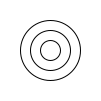Figure 14: Simple archery target

For bonus credit add a stand so we can place the target on a range, as shown in fig. 15.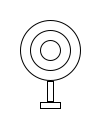Figure 15: Archery target with a stand

The simplest solution is to create three concentric circles using the `on` method:

``````Image
.circle(20)
.on(Image.circle(40))
.on(Image.circle(60))``````

For the extra credit we can create a stand using two rectangles:

``````Image
.circle(20)
.on(Image.circle(40))
.on(Image.circle(60))
.above(Image.rectangle(6, 20))
.above(Image.rectangle(20, 6))``````

### 3.5.2 Stay on Target

Colour your target red and white, the stand in brown (if applicable), and some ground in green. See fig. 16 for an example.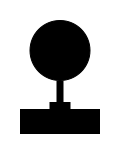Figure 16: Colour archery target

The trick here is using parentheses to control the order of composition. The `fillColor()`, `strokeColor()`, and `strokeWidth()` methods apply to a single image—we need to make sure that image comprises the correct set of shapes:

``````Image
.circle(20).fillColor(Color.red)
.on(Image.circle(40).fillColor(Color.white))
.on(Image.circle(60).fillColor(Color.red))
.above(Image.rectangle(6, 20).fillColor(Color.brown))
.above(Image.rectangle(20, 6).fillColor(Color.brown))
.above(Image.rectangle(80, 25).noStroke.fillColor(Color.green))``````

# 4 Writing Larger Programs

We’re getting to the point where it’s inconvenient to type programs into the console. In this chapter we’ll learn about two tools for writing larger programs:

• saving programs to a file so we don’t have to type code over and over again;
• giving names to values so we can reuse them.

If you run the examples from the SBT console within Doodle they will just work. If not, you will need to start your code with the following imports to make Doodle available.

``````import doodle.core._
import doodle.image._
import doodle.image.syntax._
import doodle.image.syntax.core._
import doodle.java2d._``````

## 4.1 Working Within the Console

Your text editor or IDE will allow you to save code to a file, but we need to save files in the right place so the Scala compiler can find them. If you’re working from the Doodle template you should save your code in the directory `src/main/scala/`.

How do we use code that we saved to a file from the console? There is a special command, that only works from the console, that allows us to run code saved in a file. This command is called `:paste`3. We follow `:paste` with the name of the file we want to run. For example, if we save in the file `src/main/scala/Example.scala` the expression

``Image.circle(100).fillColor(Color.paleGoldenrod).strokeColor(Color.indianRed)``

we can then run this code by writing at the console

``````:paste src/main/scala/Example.scala
// res0: doodle.core.Image = ContextTransform(<function1>,ContextTransform(<function1>,Circle(100.0)))``````

Note the value has been given the name `res0` in the example above. If you’re following along, the name in your console might end with a different number depending on what you’ve already typed into the console. We can draw the image by evaluating `res0.draw` (or the correct name for your console).

### 4.1.1 Tips for Using the Console

Here are a few tips for using the console more productively:

• If you press the up arrow you’ll get the last thing you typed into the console. Handy to avoid having to type in those long file names over and over again! You can press up multiple times to go through the history of your interactions at the console.

• You can press the `Tab` key to get the console to suggest completions for code, but unfortunately not file names, you’re typing. For example, if you type `Stri` and then press `Tab`, the console will show possible completions. Type `Strin` and the console will complete `String` for you.

Once we start saving code to a file, we’ll likely find the compiler doesn’t like our code next time we start SBT. Read the next section to see how we can fix this problem.

## 4.2 Coding Outside the Console

The code we’ve been writing inside the console will cause problems running outside the console. For example, put the following code into `Example.scala` in the `src/main/scala`.

``Image.circle(100).fillColor(Color.paleGoldenrod).strokeColor(Color.indianRed)``

Now restart SBT and try to enter the console. You should see an error similar to

``````[error] src/main/scala/Example.scala:1: expected class or object definition
[error] circle(100) fillColor Color.paleGoldenrod strokeColor Color.indianRed
[error] ^
[error] one error found``````

You’ll see something similar if you’re using an IDE.

The problem is this:

• Scala is attempting to compile all our code before the console starts; and
• there are restrictions on code written in files that don’t apply to code written directly in the console.

We need to know about these restrictions and change how we write code in files accordingly.

The error message gives us some hint: `expected class or object definition`. We don’t yet know what a class is, but we do know about objects—all values are objects. In Scala all code in a file must be written inside an object or class. We can easily define an object by wrapping an expression like the below.

``````object Example {
Image.circle(100).fillColor(Color.paleGoldenrod).strokeColor(Color.indianRed).draw()
}``````

Now the code won’t compile for a different reason. You should see a lot of errors similar to

``````[error] doodle/shared/src/main/scala/doodle/examples/Example.scala:1: not found: value Image
[error]   Image.circle(100).fillColor(Color.paleGoldenrod).strokeColor(Color.indianRed).draw()
[error]   ^``````

The compiler is saying that we’ve used a name, `circle`, but the compiler doesn’t know what value this name refers to. It will have a similiar issue with `Color` in the code above. We’ll talk in more details about names in just a moment. Right now let’s tell the compiler where it can find the values for these names by adding some `import` statements. The name `Color` is found inside a package called `doodle.core`, and the name `circle` is within the object `Image` that is in `doodle.image`. We can tell the compiler to use all the name in `doodle.core`, and all the names in `doodle.image` by writing

``````import doodle.core._
import doodle.image._``````

There are a few other names that the compiler will need to find for the complete code to work. We can import these with the lines

``````import doodle.image.syntax._
import doodle.image.syntax.core._
import doodle.java2d._``````

We should place all these imports at the top of the file, so the complete code looks like

``````import doodle.core._
import doodle.image._
import doodle.image.syntax._
import doodle.image.syntax.core._
import doodle.java2d._

object Example {
Image.circle(100).fillColor(Color.paleGoldenrod).strokeColor(Color.indianRed).draw()
}``````

With this in place the code should compile without issue.

Now when we go to the console within SBT we can refer to our code using the name, `Example`, that we’ve given it.

``Example // draws the image``

### Exercise

If you haven’t done so already, save the code above in the file `src/main/scala/Example.scala` and check that the code compiles and you can access it from the console.

## 4.3 Names

In the previous section we introduced a lot of new concepts. In this section we’ll explore one of those concepts: naming values.

We use names to refer to things. For example, “Professeur Emile Perrot” refers to a very fragrant rose variety, while “Cherry Parfait” is a highly disease resistant variety but barely smells at all. Much ink has been spilled, and many a chin stroked, on how exactly this relationship works in spoken language. Programming languages are much more constrained, which allows us to be much more precise: names refer to values. We will sometimes say names are bound to values, or a name introduces a binding. Wherever we would write out a value we can instead use its name, if the value has a name. In other words, a name evaluates to the value it refers to. This naturally raises the question: how do we give names to values? There are several ways to do this in Scala. Let’s see a few.

### 4.3.1 Object Literals

We have already seen an example of declaring an object literal.

``````object Example {
Image.circle(100).fillColor(Color.paleGoldenrod).strokeColor(Color.indianRed).draw()
}``````

This is a literal expression, like other literals we’ve seen so far, but in this case it creates an object with the name `Example`. When we use the name `Example` in a program it evaluates to that object.

``````Example
// Example.type = Example\$@76c39258``````

Try this in the console a few times. Do you notice any difference in uses of the name? You might have noticed that the first time you entered the name `Example` a picture was drawn, but on subsequent uses this didn’t happen. The first time we use an object’s name the body of the object is evaluated and the object is created. On subsequent uses of the name the object already exists and is not evaluated again. We can tell there is a difference in this case because the expression inside the object calls the `draw` method. If we replaced it with something like `1 + 1` (or just dropped the call to `draw`) we would not be able to tell the difference. We’ll have plenty more to say about this in a later chapter.

We might wonder about the type of the object we’ve just created. We can ask the console about this.

``````:type Example
// Example.type``````

The type of `Example` is `Example.type`, a unique type that no other value has.

### 4.3.2`val` Declarations

Declaring an object literal mixes together object creation and defining a name. It would be useful if we could separate the two, so we could give a name to a pre-existing object. A `val` declaration allows us to do this.

We use `val` by writing

``val <name> = <value>``

replacing `<name>` and `<value>` with the name and the expression evaluating to the value respectively. For example

``````val one = 1
val anImage = Image.circle(100).fillColor(Color.red)``````

These two declarations define the names `one` and `anImage`. We can use these names to refer to the values in later code.

``````one
// res0: Int = 1
anImage
// res1: Image = FillColor(
//   Circle(100.0),
//   RGBA(
//     UnsignedByte(127),
//     UnsignedByte(-128),
//     UnsignedByte(-128),
//     Normalized(1.0)
//   )
// )``````

### 4.3.3 Declarations

We’ve talked about declarations and definitions above. It’s now time to be precise about what these terms mean, and to look in a bit more depth at the differences between `object` and `val`.

We already know about expressions. They are a part of a program that evaluates to a value. A declaration or definition is another part of a program, but do not evaluate to a value. Instead they give a name to something—not always to a value as you can declare types in Scala, though we won’t spend much time on this. Both `object` and `val` are declarations.

One consequence of declarations being separate from expressions is we can’t write program like

``val one = ( val aNumber = 1 )``

because `val aNumber = 1` is not an expression and thus does not evaluate to a value.

We can however write

``````val aNumber = 1
// aNumber: Int = 1
val one = aNumber
// one: Int = 1``````

### 4.3.4 The Top-Level

It seems a bit unsatisfactory to have both `object` and `val` declarations, as they both give names to values. Why not just have `val` for declaring names, and make `object` just create objects without naming them? Can you declare an object literal without a name?

No, Scala doesn’t allow us to do this. For example, we can’t write

``object {}``

We have to give a name to any object literal we create.

Scala distinguishes between what is called the top-level and other code. Code at the top-level is code that doesn’t have any other code wrapped around. In other words it is something we can write in a file and Scala will compile without having to wrap it in an `object`.

We’ve seen that expressions aren’t allowed at the top-level. Neither are `val` definitions. Object literals, however, are.

This distinction is a bit annoying. Some other languages don’t have this restriction. In Scala’s case it comes about because Scala builds on top of the Java Virtual Machine (JVM), which was designed to run Java code. Java makes a distinction between top-level and other code, and Scala is forced to make this distinction to work with the JVM. The Scala console doesn’t make this top-level distinction (we can think of everything written in the console being wrapped in some object) which can lead to confusion when we first start using Scala.

If an object literal is allowed at the top-level, but a `val` definition is not, does this mean we can declare a `val` inside an object literal? If we can declare a `val` inside an object literal, can we later refer to that name?

We sure can!

We can put a `val` inside an object literal like so:

``````object Example {
val hi = "Hi!"
}``````

We can then refer to it using the `.` syntax we’ve been using already.

``````Example.hi
// res4: String = "Hi!"``````

Note that we can’t use `hi` on it’s own

``````hi
// error: not found: value hi``````

We have to tell Scala we want to refer to the name `hi` defined inside the object `Example`.

### 4.3.5 Scope

If you did the last exercise (and you did, didn’t you?) you’ll have seen that a name declared inside an object can’t be used outside the object without also referring to the object that contains the name. Concretely, if we declare

``````object Example {
val hi = "Hi!"
}``````

we can’t write

``````hi
// error: not found: value hi``````

We must tell Scala to look for `hi` inside `Example`.

``````Example.hi
// res8: String = "Hi!"``````

We say that a name is visible in the places where it can be used without qualification, and we call the places where a name is visible its scope. So using our fancy-pants new terminology, `hi` is not visible outside of `Example`, or alternatively `hi` is not in scope outside of `Example`.

How do we work out the scope of a name? The rule is fairly simple: a name is visible from the point it is declared to the end of the nearest enclosing braces (braces are `{` and `}`). In the example above `hi` is enclosed by the braces of `Example` and so is visible there. It’s not visible elsewhere.

We can declare object literals inside object literals, which allows us to make finer distinctions about scope. For example in the code below

``````object Example1 {
val hi = "Hi!"

object Example2 {
val hello = "Hello!"
}
}``````

`hi` is in scope in `Example2` (`Example2` is defined within the braces that enclose `hi`). However the scope of `hello` is restricted to `Example2`, and so it has a smaller scope than `hi`.

What happens if we declare a name within a scope where it is already declared? This is known as shadowing. In the code below the definition of `hi` within `Example2` shadows the definition of `hi` in `Example1`

``````object Example1 {
val hi = "Hi!"

object Example2 {
val hi = "Hello!"
}
}``````

Scala let’s us do this, but it is generally a bad idea as it can make code very confusing.

We don’t have to use object literals to create new scopes. Scala allows us to create a new scope just about anywhere by inserting braces. So we can write

``````object Example {
val good = "Good"

// Create a new scope
{
val morning = good ++ " morning"
val toYou = morning ++ " to you"
}

val day = good ++ " day, sir!"
}``````

`morning` (and `toYou`) is declared within a new scope. We have no way to refer to this scope from the outside (it has no name) so we cannot refer to `morning` outside of the scope where it is declared. If we had some secrets that we didn’t want the rest of the program to know about this is one way we could hide them.

The way nested scopes work in Scala is called lexical scoping. Not all languages have lexical scoping. For example, Ruby and Python do not, and Javascript has only recently acquired lexical scoping. It is the authors’ opinion that creating a language without lexical scope is an idea on par with eating a bushel of Guatemalan insanity peppers and then going to the toilet without washing your hands.

### Exercises

Test your understanding of names and scoping by working out the value of `answer` in each case below.

``````val a = 1
val b = 2
val answer = a + b``````

A simple example to get started with. `answer` is `1 + 2`, which is `3`.

``````object One {
val a = 1

object Two {
val a = 3
val b = 2
}

object Answer {
val answer = a + Two.b
}
}``````

Another simple example. `answer` is `1 + 2`, which is `3`. `Two.a` is not in scope where `answer` is defined.

``````object One {
val a = 5
val b = 2

object Answer {
val a = 1
val answer = a + b
}
}``````

Here `Answer.a` shadows `One.a` so `answer` is `1 + 2`, which is `3`.

``````object One {
val a = 1
val b = a + 1
val answer = a + b
}``````

This is perfectly fine. The expression `a + 1` on the right hand side of the declaration of `b` is an expression like any other so `answer` is `3` again.

``````object One {
val a = 1

object Two {
val b = 2
}

val answer = a + b
}``````

This code doesn’t compile as `b` is not in scope where `answer` is declared.

``````object One {
val a = b - 1
val b = a + 1

val answer = a + b
}``````

Trick question! This code doesn’t work. Here `a` and `b` are defined in terms of each other which leads to a circular dependency that can’t be resolved.

## 4.4 Abstraction

We’ve learned a lot about names in the previous section. If we want to use fancy programmer words, we could say that names abstract over expressions. This usefully captures the essence of what defining names does, so let’s decode the programmer-talk.

To abstract means to remove unnecessary details. For example, numbers are an abstraction. The number “one” is never found in nature as a pure concept. It’s always one object, such as one apple, or one copy of Creative Scala. When doing arithmetic the concept of numbers allows us to abstract away the unnecessary detail of the exact objects we’re counting and manipulate the numbers on their own.

Similarly a name stands in for an expression. An expression tells us how to construct a value. If that value has a name then we don’t need to know anything about how the value is constructed. The expression can have arbitrary complexity, but we don’t have to care about this complexity if we just use the name. This is what it means when we say that names abstract over expressions. Whenever we have an expression we can substitute a name that refers to the same value.

Abstraction makes code easier to read and write. Let’s take as an example creating a sequence of boxes like shown in fig. 17.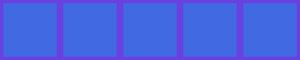Figure 17: Five boxes filled with Royal Blue

We can write out a single expression that creates the picture.

``````Image.rectangle(40, 40)
.strokeWidth(5.0)
.strokeColor(Color.royalBlue.spin(30.degrees))
.fillColor(Color.royalBlue)
.beside(
Image.rectangle(40, 40)
.strokeWidth(5.0)
.strokeColor(Color.royalBlue.spin(30.degrees))
.fillColor(Color.royalBlue)
).beside(
Image.rectangle(40, 40)
.strokeWidth(5.0)
.strokeColor(Color.royalBlue.spin(30.degrees))
.fillColor(Color.royalBlue)
).beside(
Image.rectangle(40, 40)
.strokeWidth(5.0)
.strokeColor(Color.royalBlue.spin(30.degrees))
.fillColor(Color.royalBlue)
).beside(
Image.rectangle(40, 40)
.strokeWidth(5.0)
.strokeColor(Color.royalBlue.spin(30.degrees))
.fillColor(Color.royalBlue)
)``````

In this code it is difficult to see the simple pattern within. Can you really tell at a glance that all the rectangles are exactly the same? If we make the abstraction of naming the basic box the code becomes much easier to read.

``````val box =
Image.rectangle(40, 40)
.strokeWidth(5.0)
.strokeColor(Color.royalBlue.spin(30.degrees))
.fillColor(Color.royalBlue)

box.beside(box).beside(box).beside(box).beside(box)``````

Now we can easily see how the box is made, and easily see that the final picture is that box repeated five times.

### Exercises

#### Archery Again

Let’s return to the archery target we created in an earlier chapter, shown in fig. 18.Figure 18: The Archery Target

Last time we created the image we didn’t know how to name values, so we can to write one large expression. This time around, give the components of the image names so that it is easier for someone else to understand how the image is constructed. You’ll have to use your own taste to decide what parts should be named and what parts don’t warrant names of their own.

We decided to name the target, stand, and ground, as shown below. This makes is clear how the final image is constructed. Naming more components seemed to us that it would not aid comprehension.

``````val coloredTarget =
(
Image.circle(10).fillColor(Color.red) on
Image.circle(20).fillColor(Color.white) on
Image.circle(30).fillColor(Color.red)
)

val stand =
Image.rectangle(6, 20) above Image.rectangle(20, 6).fillColor(Color.brown)

val ground =
Image.rectangle(80, 25).strokeWidth(0).fillColor(Color.green)

val image = coloredTarget above stand above ground``````

#### Streets Ahead

For a more compelling use of names, create a street scene like that shown in fig. 19. By naming the individual components of the image you should be able to avoid a great deal of repetition.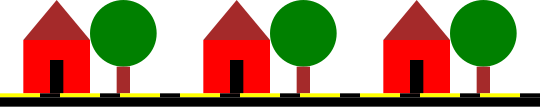Figure 19: A Street Scene

Here’s our solution. As you can see, by breaking the scene down into smaller components we were able to write relatively little code.

``````val roof = Image.triangle(50, 30) fillColor Color.brown

val frontDoor =
(Image.rectangle(50, 15) fillColor Color.red) above (
(Image.rectangle(10, 25) fillColor Color.black) on
(Image.rectangle(50, 25) fillColor Color.red)
)

val house = roof above frontDoor

val tree =
(
(Image.circle(25) fillColor Color.green) above
(Image.rectangle(10, 20) fillColor Color.brown)
)

val streetSegment =
(
(Image.rectangle(30, 3) fillColor Color.yellow) beside
(Image.rectangle(15, 3) fillColor Color.black) above
(Image.rectangle(45, 7) fillColor Color.black)
)

val street = streetSegment beside streetSegment beside streetSegment

val houseAndGarden =
(house beside tree) above street

val image = (
houseAndGarden beside
houseAndGarden beside
houseAndGarden
) strokeWidth 0``````

## 4.5 Packages and Imports

When we changed our code to compile we had to add many import statements to it. In this section we learn about them.

We’ve seen that one name can shadow another. This can cause problems in larger programs as many parts of a program many want to put a common name to different uses. We can create scopes to hide names from the outside, but we must still deal with names defined at the top-level.

We have the same problem in natural language. For example, if both your brother and friend were called “Ziggy” you would have to qualify which one you meant when you used their name. Perhaps you could tell from context, or perhaps your friend was “Ziggy S” and your brother was just “Ziggy”.

In Scala we can use packages to organise names. A package creates a scope for names defined at the top-level. All top-level names within the same package are defined in the same scope. To bring names in a package into another scope we must import them.

Creating a package is simple: we write

``package <name>``

at the top of the file, replace `<name>` with the name of our package.

When we want to use names defined in a package we use an `import` statement, specifying the package name followed by `_` for all names, or the just the name we want if we only want one or a few names.

Here’s an example.

You can’t define packages in the console. To get the following code to work you must put the code within the package `example` into a file and compile it.

Let’s start by defining some names within a package.

``````package example

object One {
val one = 1
}

object Two {
val two = 2
}

object Three {
val three = 3
}``````

Now to bring these names into scope we must import them. We could import just one name.

``````import example.One

One.one``````

Or both `One` and `Two`.

``````import example.{One, Two}

One.one + Two.two``````

Or all the names in `example`.

``````import example._

One.one + Two.two + Three.three``````

In Scala we can also import just about anything that defines a scope, including objects. So the following code brings `one` into scope.

``````import example.One._

one``````

### 4.5.1 Package Organisation

Packages stop top-level names from colliding, but what about collisions between package names? It’s common to organise packages in a hierarchy, which helps to avoid collisions. For example, in Doodle the package `core` is defined within the package `doodle`. When we use the statement

``import doodle.core._``

we’re indicating we want the package `core` within the package `doodle`, and not some other package that might be called `core`.

# 5 The Substitution Model of Evaluation

We need to build a mental model of how Scala expressions are evaluated so we can understand what our programs are doing. We’ve been getting by with an informal model so far. In this section we make our model a bit more formal by learning about the substitution model of evaluation. Like many things in programming we’re using some fancy words for a simple concept. In this case you’ve probably already learned about substitution in high school algebra, and we’re just taking those ideas into a new context.

If you run the examples from the SBT console within Doodle they will just work. If not, you will need to start your code with the following imports to make Doodle available.

``````import doodle.core._
import doodle.image._
import doodle.image.syntax._
import doodle.image.syntax.core._
import doodle.java2d._``````

## 5.1 Substitution

Substitution says that wherever we see an expression we can replace it with the value it evaluates to. For example, where we see

``1 + 1``

we can replace it with `2`. This in turn means when we see a compound expression such as

``(1 + 1) + (1 + 1)``

we can substitute `2` for `1 + 1` giving

``2 + 2``

which evaluates to `4`.

This type of reasoning is what we do in high school algebra when we simplify an expression. Naturally computer science has fancy words for this process. In addition to substitution, we can call this reducing an expression, or equational reasoning.

Substitution gives us a way to reason about our programs, which is another way of saying “working out what they do”. We can apply substitution to just about any expression we’ve seen so far. It’s easier to use examples that work with numbers and strings, rather than images, here so we’ll return to an example we saw in an earlier chapter:

``1 + ("Moonage daydream".indexOf("N"))``

In the previous example we were a bit fast-and-loose. Here we will be a bit more precise to illustrate the steps the computer would have to go through. We are trying to emulate the computer, after all.

The expression containing the `+` consists of two sub-expressions, `1` and `("Moonage daydream".indexOf("N"))`. We have to decide which to evaluate first: the left or the right. Let’s arbitrarily choose the right sub-expression (we’ll return to this choice later.)

The sub-expression `("Moonage daydream".indexOf("N"))` again consists of two sub-expressions, `"Moonage daydream"` and `"N"`. Let’s again evaluate the right-hand first, remembering that literal expressions are not values so they must be evaluated.

The literal `"N"` evaluates to the value `"N"`. To avoid this confusion let’s write the value as `|"N"|`. Now we can substitute the value for the expression given in our first steps

``1 + ("Moonage daydream".indexOf(|"N"|))``

Now we can evaluate the left-hand side of the sub-expression, substituting the literal expression `"Moonage daydream"` with its value `|"Moonage daydream"|`. This gives us

``1 + (|"Moonage daydream"|.indexOf(|"N"|))``

Now we’re in a position to evaluate the entire expression `(|"Moonage daydream"|.indexOf(|"N"|))`, which evaluates to `|-1|` (again differentiating the integer value from the literal expression by using a vertical bar). Once again we perform substitution and now we have

``1 + |-1|``

Now we should evaluate the left-hand side literal `1`, giving `|1|`. Perform substitution and we get

``|1| + |-1|``

Now we can evaluate the entire expression, giving

``|0|``

We can ask Scala to evaluate the whole expression to check our work.

``````1 + ("Moonage daydream".indexOf("N"))
// res4: Int = 0``````

Correct!

There are some observations we might make at this point:

• doing substitution rigorously like a computer might involve a lot of steps;
• the shortcut evaluation you probably did in your head probably got to the correct answer; and
• our seemingly arbitrary choice to do evaluation from right-to-left got us the correct answer.

Did we somehow manage to choose the same substitution order that Scala uses (no we didn’t, but we haven’t investigated this yet) or does it not really matter what order we choose? When exactly can we take shortcuts and still reach the right result, like we did in the first example with addition? We will investigate these questions in just a moment, but first let’s talk about how substitution works with names.

### 5.1.1 Names

The substitution rule for names is to substitute the name with the value it refers to. We’ve already been using this rule implicitly. Now we’re just formalising it.

For example, given the code

``````val name = "Ada"
name ++ " " ++ "Lovelace"``````

we can apply substitution to get

``"Ada" ++ " " ++ "Lovelace"``

which evaluates to

``"Ada Lovelace"``

We can use names to be a bit more formal with our substitution process. Returning to our first example

``1 + 1``

we can give this expression a name:

``val two = 1 + 1``

When we see a compound expression such as

``(1 + 1) + (1 + 1)``

substitution tells us we can substitute `two` for `1 + 1` giving

``two + two``

Remember when we worked through the expression

``1 + ("Moonage daydream".indexOf("N"))``

we broke it into sub-expressions which we then evaluated and substituted. Using words, this was quite convoluted. With a few `val` declarations we can make this both more compact and easier to see. Here’s the same expression broken into it’s components.

``````val a = 1
val b = "Moonage daydream"
val c = "N"
val d = b.indexOf(c)
val e = a + d``````

If we (at this point, arbitrarily) define that evaluation occurs from top-to-bottom we can experiment with different ordering to see what difference they make.

For example,

``````val c = "N"
val b = "Moonage daydream"
val a = 1
val d = b.indexOf(c)
val e = a + d``````

achieves the same result as before. However we can’t use

``````val e = a + d
val a = 1
val b = "Moonage daydream"
val c = "N"
val d = b.indexOf(c)``````

because `e` depends on `a` and `d`, and in our top-to-bottom ordering `a` and `d` have yet to be evaluated. We might rightly claim that this is a bit silly to even attempt. The complete expression we’re trying to evaluate is `e` but `a` to `d` are sub-expressions of `e`, so of course we have to evaluate the sub-expressions before we evaluate the expression.

## 5.2 Order of Evaluation

We’re now ready to tackle the question of order-of-evaluation. We might wonder if the order of evaluation even matters? In the examples we’ve looked at so far the order doesn’t seem to matter, except for the issue that we cannot evaluate an expression before it’s sub-expressions.

To investigate these issues further we need to introduce a new concept. So far we have almost always dealt with pure expressions. These are expressions that we can freely substitute in any order without issue4.

Impure expressions are those where the order of evaluation matters. We have already used one impure expression, the method `draw`. If we evaluate

``````Image.circle(100).draw
Image.rectangle(100, 50).draw``````

and

``````Image.rectangle(100, 50).draw
Image.circle(100).draw``````

the windows containing the images will appear in different orders. Hardly an exciting difference, but it is a difference, which is the point.

The key distinguishing feature of impure expressions is that their evaluation causes some change that we can see. For example, evaluating `draw` causes an image to be displayed. We call these observable changes side effects, or just effects for short. In a program containing side effects we cannot freely use substitution. However we can use side effects to investigate the order of evaluation. Our tool for doing so will be the `println` method.

The `println` method displays text on the console (a side effect) and evaluates to unit. Here’s an example:

``````println("Hello!")
// Hello!``````

The side-effect of `println`—printing to the console—gives us a convenient way to investigate the order of evaluation. For example, the result of running

``````println("A")
// A
println("B")
// B
println("C")
// C``````

indicates to us that expressions are evaluated from top to bottom. Let’s use `println` to investigate further.

### Exercises

#### No Substitute for Println

In a pure program we can give a name to any expression and substitute any other occurrences of that expression with the name. Concretely, we can rewrite

``(2 + 2) + (2 + 2)``

to

``````val a = (2 + 2)
a + a``````

and the result of the program doesn’t change.

Using `println` as an example of an impure expression, demonstrates that this is not the case for impure expressions, and hence we can say that impure expressions, or side effects, break substitution.

Here is a simple example that illustrates this. The following two programs are observably different.

``````println("Happy birthday to you!")
// Happy birthday to you!
println("Happy birthday to you!")
// Happy birthday to you!
println("Happy birthday to you!")
// Happy birthday to you!

val happy = println("Happy birthday to you!")
// Happy birthday to you!
happy
happy
happy``````

Therefore we cannot freely use substitution in the presence of side effects, and we must be aware of the order of evaluation.

#### Madness to our Methods

When we introduced scopes we also introduced block expressions, though we didn’t call them that at the time. A block is created by curly braces (`{}`). It evaluates all the expressions inside the braces. The final result is the result of the last expression in the block.

``````// Evaluates to three
{
val one = 1
val two = 2
one + two
}
// res12: Int = 3``````

We can use block expressions to investigate the order in which method parameters are evaluated, by putting `println` expression inside a block that evaluates to some other useful value.

For example, using `Image.rectangle` or `Color.hsl` and block expressions, we can determine if Scala evaluates method parameters in a fixed order, and if so what that order is.

Note that you can write a block compactly, on one line, by separating expressions with semicolons (`;`). This is generally not good style but might be useful for these experiments. Here’s an example.

``````// Evaluates to three
{ val one = 1; val two = 2; one + two }
// res13: Int = 3``````

The following code demonstrates that method parameters are evaluated from left to right.

``````Color.hsl(
{
println("a")
0.degrees
},
{
println("b")
1.0
},
{
println("c")
1.0
}
)
// a
// b
// c
// res14: Color = HSLA(
//   Angle(0.0),
//   Normalized(1.0),
//   Normalized(1.0),
//   Normalized(1.0)
// )``````

We can write this more compactly as

``````Color.hsl({ println("a"); 0.degrees },
{ println("b"); 1.0 },
{ println("c"); 1.0 })
// a
// b
// c
// res15: Color = HSLA(
//   Angle(0.0),
//   Normalized(1.0),
//   Normalized(1.0),
//   Normalized(1.0)
// )``````

#### The Last Order

In what order are Scala expressions evaluated? Perform whatever experiments you need to determine an answer to this question to your own satisfaction. You can reasonably assume that Scala uses consistent rules across all expressions. There aren’t special cases for different expressions.

We’ve already seen that expressions are evaluated from top-to-bottom, and method parameters are evaluated from left-to-right. We might want to check that expressions are in general evaluated left-to-right. We can show this fairly easily.

``````{ println("a"); 1 } + { println("b"); 2 } + { println("c"); 3}
// a
// b
// c
// res16: Int = 6``````

So in conclusion we can say that Scala expressions are evaluated from top-to-bottom and left-to-right.

## 5.3 Local Reasoning

We’ve seen that the order of evaluation is only really important when we have side effects. For example, if the following expressions produce side effects

``````disableWarheads()
launchTheMissles()``````

we really want to ensure that the expressions are evaluated top to bottom so the warheads are disabled before the missles are launched.

All useful programs must have some effect, because effects are how the program interacts with the outside world. The effect might just be printing out something when the program has finished, but it’s still there. Minimising side effects is a key goal of functional programming so we will spend a few more words on this topic.

Substitution is really easy to understand. When the order of evaluation doesn’t matter it means any other code cannot change the meaning of the code we’re looking at. `1 + 1` is always `2`, no matter what other code we have in our program, but the effect of `launchTheMissles()` depends on whether we have already disabled the warheads or not.

The upshot of this is that pure code can be understood in isolation. Since no other code can change its meaning, if we’re only interested in one fragment we can ignore the rest of the code. The meaning of impure code, on the other hand, depends on all the code that will have run before it is evaluated. This property is known as local reasoning. Pure code has it, but impure code does not.

As programs get larger it becomes harder and harder to keep all the details in our head. Since the size of our head is a fixed quantity the only solution is to introduce abstraction. Remember that an abstraction is the removal of irrelevant details. Pure code is the ultimate abstraction, because it tells us that everything else is an irrelevant detail. This is one of the properties that gets functional programmers really excited: the ability to make large programs understandable. Functional programming doesn’t mean avoiding effects, because all useful programs have effects. It does, however, mean controlling effects so the majority of the code can be reasoned about using the simple model of substitution.

### 5.3.1 The Meaning of Meaning

So far, we’ve talked a lot about the meaning of code, where we’ve taken “meaning” to mean to the result it evaluates to, and perhaps the side effects it performs.

In substitution, the meaning of a program is exactly what it evaluates to. Thus two programs are equal if they evaluate to the same result. This is precisely why side effects break substitution: the substitution model has no notion of side effects and therefore cannot distinguish two programs that differ by their effects.

There are other ways in which programs can differ. For example, one program may take longer than another to produce the same result. Again, substitution does not distinguish them.

Substitution is an abstraction, and the details it throws away are everything except for the value. Side effects, time, and memory usage are all irrelevant to substitution, but perhaps not to the people writing or running the program. There is a tradeoff here. We can employ richer models that capture more of these details, but they are much harder to work with. For most people most of the time substitution makes the right tradeoff of being dead simple to use while still being useful.

# 6 Methods

We’ve already used methods—methods are the way we interact with objects. In this chapter we’ll learn how to write our own methods.

Names allow us to abstract over expressions. Methods allow us to abstract over and generalise expressions. By generalisation we mean the ability to express a group of related things, in this case expressions. A method captures a template for an expression, and allows the caller to fill in parts of that template by passing the method parameters.

If you run the examples from the SBT console within Doodle they will just work. If not, you will need to start your code with the following imports to make Doodle available.

``````import doodle.core._
import doodle.image._
import doodle.image.syntax._
import doodle.image.syntax.core._
import doodle.java2d._``````

## 6.1 Methods

In a previous chapter we created the image shown in fig. 20 using the programFigure 20: Five boxes filled with Royal Blue

``````val box =
Image.rectangle(40, 40).
strokeWidth(5.0).
strokeColor(Color.royalBlue.spin(30.degrees)).
fillColor(Color.royalBlue)

box beside box beside box beside box beside box``````

Imagine we wanted to change the color of the boxes. Right now we would have to write out the expression again for each different choice of color.

``````val paleGoldenrod = {
val box =
Image.rectangle(40, 40).
strokeWidth(5.0).
strokeColor(Color.paleGoldenrod.spin(30.degrees)).
fillColor(Color.paleGoldenrod)

box beside box beside box beside box beside box
}

val lightSteelBlue = {
val box =
Image.rectangle(40, 40).
strokeWidth(5.0).
strokeColor(Color.lightSteelBlue.spin(30.degrees)).
fillColor(Color.lightSteelBlue)

box beside box beside box beside box beside box
}

val mistyRose = {
val box =
Image.rectangle(40, 40).
strokeWidth(5.0).
strokeColor(Color.mistyRose.spin(30.degrees)).
fillColor(Color.mistyRose)

box beside box beside box beside box beside box
}``````

This is tedious. Each expression only differs in a minor way. It would be nice if we could capture the general pattern and allow the color to vary. We can do exactly this by declaring a method.

``````def boxes(color: Color): Image = {
val box =
Image.rectangle(40, 40).
strokeWidth(5.0).
strokeColor(color.spin(30.degrees)).
fillColor(color)

box beside box beside box beside box beside box
}

// Create boxes with different colors
boxes(Color.paleGoldenrod)
boxes(Color.lightSteelBlue)
boxes(Color.mistyRose)``````

Try this yourself to see that you get the same result using the method as you did writing everything out by hand.

Now that we’ve seen an example of declaring a method, we need to explain the syntax of methods. Next, we’ll look at how to write methods, the semantics of method calls, and how they work in terms of substitution.

## 6.2 Method Syntax

We’ve already seen an example of declaring a method.

``````def boxes(color: Color): Image = {
val box =
Image.rectangle(40, 40).
strokeWidth(5.0).
strokeColor(color.spin(30.degrees)).
fillColor(color)

box beside box beside box beside box beside box
}``````

Let’s use this as a model for understanding the syntax of declaring a method. The first part is the keyword `def`. A keyword is a special word that indicates something important to the Scala compiler—in this case that we’re going to declare a method. We’re already seen the `object` and `val` keywords.

The `def` is immediately followed by the name of the method, in this case `boxes`, in the same way that `val` and `object` are immediately followed by the name they declare. Like a `val` declaration, a method declaration is not a top-level declaration and must be wrapped in an `object` declaration (or other top-level declaration) when written in a file.

Next we have the method parameters, defined in brackets (`()`). The method parameters are the parts that the caller can “plug-in” to the expression that the method evaluates. When declaring method parameters we must give them both a name and a type. A colon (`:`) separates the name and the type. We haven’t had to declare types before. Most of the time Scala will work out the types for us, a process known as type inference. Type inference, however, cannot infer the type of method parameters so we must provide them.

After the method parameters comes the result type. The result type is the type of the value the method evaluates to when it is called. Unlike parameter types Scala can infer the result type, but it is good practice to include it and we will do so throughout Creative Scala.

Finally, the body expression of the method calculates the result of calling the method. A body can be a block expression, as in `boxes` above, or just a single expression.

#### Method Declaration Syntax

The syntax for a method declaration is

``````def methodName(param1: Param1Type, ...): ResultType =
bodyExpression``````

where

• `methodName` is the name of the method;
• the optional `param1 : Param1Type, ...` are one or more pairs of parameter name and parameter type;
• the optional `ResultType` is the type of the result of calling the method; and
• `bodyExpression` is the expression that is evaluated to yield the result of calling the method.

### Exercises

Let’s practice declaring methods by writing some simple examples.

#### Square

Write a method `square` that accepts an `Int` argument and returns the `Int` square of it’s argument. (Squaring a number is multiplying it by itself.)

The solution is

``````def square(x: Int): Int =
x * x``````

We can arrive at the solution by the following steps.

We’re given the name (`square`), the type of the parameter (`Int`), and the type of the result (`Int`). From this we can write the method skeleton

``````def square(x: Int): Int =
???``````

where we have chosen `x` as the name of the parameter. This is a fairly arbitrary choice. Where there is no meaningful name you often see one-letter names such as `x`, `v`, or `i` used.

By the way this is valid code. Enter it into the console and see! What happens if you call `square` when it’s defined like so?

Now we need to complete the body. We’ve been told that squaring is multiplying a number by itself, so `x * x` is what we replace the `???` with. We don’t need to wrap this in braces as there is only a single expression in the body.

#### Halve

Write a method `halve` that accepts a `Double` argument and returns the `Double` that is half of it’s argument.

``````def halve(x: Double): Double =
x / 2.0``````

We can follow the same process as for `square` above to arrive at the solution.

## 6.3 Method Semantics

Now that we know how to declare methods, let’s turn to the semantics. How do we understand a method call in terms of our substitution model?

We already know we can substitute a method call with the value it evaluates to. However we need a more fine-grained model so we can work out what this value will be. Our extended model is as follows: when we see a method call we will create a new block and within this block: bind the parameters to the respective expressions given in the method call and substitute the method body.

We can then apply substitution as usual.

Let’s see a simple example. Given the method

``````def square(x: Int): Int =
x * x``````

we can expand the method call

``square(2)``

by introducing a block

``````{
square(2)
}``````

binding the parameter `x` to the expression `2`

``````{
val x = 2
square(2)
}``````

and substituting the method body

``````{
val x = 2
x * x
}``````

We can now perform substitution as usual giving

``````{
2 * 2
}``````

and finally

``````{
4
}``````

Once again we see that substitution is involved but no single step was particularly difficult.

### Exercise

Last time we looked at substitution we spent a lot of time investigating order of evaluation. In the description above we have decided that a method’s arguments are evaluated before the body is evaluated. This is not the only possibility. We could, for example, evaluate the method’s arguments only at the point they are needed. This could save us some time if a method doesn’t use one of its parameters. By using our old friend `println`, determine when method parameters are evaluated in Scala.

The following program demonstrates that parameters are evaluated before the method body.

``````def example(a: Int, b: Int): Int = {
println("In the method body!")
a + b
}

example({ println("a"); 1 }, { println("b"); 2 })
// a
// b
// In the method body!
// res6: Int = 3``````

The alternative we described above is used by some languages, most notably Haskell, and is known as lazy or non-strict evaluation.

## 6.4 Conclusions

In this chapter, we learned how to write our own simple methods and we saw how to use the substitution model of evaluation to understand method calls.

We saw that methods both abstract over expressions, in the same way as names, and also generalize over expressions, allowing us to represent a group of related expressions with one name.

We wrote some interesting methods, but we still have more repeated code than is desirable (think about the repeated calls to `box` and `circle` in the exercises.) In the next chapter, we will learn how we can generalize over this using structural recursion over the natural numbers.

# 7 Structural Recursion

In this chapter we see our first major pattern for structuring computations: structural recursion over the natural numbers. That’s quite a mouthful, so let’s break it down:

• By a pattern, we mean a way of writing code that is useful in lots of different contexts. We’ll encounter structural recursion in many different situations throughout this book.

• By the natural numbers we mean the whole numbers 0, 1, 2, and upwards.

• By recursion we mean something that refers to itself. Structural recursion means a recursion that follows the structure of the data it is processing. If the data is recursive (refers to itself) then the structural recursion will also refer to itself. We’ll see in more detail what this means in a moment.

If you run the examples from the SBT console within Doodle they will just work. If not, you will need to start your code with the following imports to make Doodle available.

``````import doodle.core._
import doodle.image._
import doodle.image.syntax._
import doodle.image.syntax.core._
import doodle.java2d._``````

## 7.1 A Line of Boxes

Let’s start with an example, drawing a line or row of boxes as in fig. 21.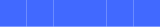Figure 21: Five boxes filled with Royal Blue

Let’s define a box to begin with.

``val aBox = Image.square(20).fillColor(Color.royalBlue)``

Then one box in a row is just

``val oneBox = aBox``

If we want to have two boxes side by side, that is easy enough.

``val twoBoxes = aBox.beside(oneBox)``

Similarly for three.

``val threeBoxes = aBox.beside(twoBoxes)``

And so on for as many boxes as we care to create.

You might think this is an unusual way to create these images. Why not just write something like this, for example?

``val threeBoxes = aBox.beside(aBox).beside(aBox)``

These two definitions are equivalent. We’ve chosen to write later images in terms of earlier ones to emphasise the structure we’re dealing with, which is building up to structural recursion.

Writing images in this way could get very tedious. What we’d really like is some way to tell the computer the number of boxes we’d like. More technically, we would like to abstract over the expressions above. We learned in the previous chapter that methods abstract over expressions, so let’s try to write a method to solve this problem.

We’ll start by writing a method skeleton that defines, as usual, what goes into the method and what it evaluates to. In this case we supply an `Int` `count`, which is the number of boxes we want, and we get back an `Image`.

``````def boxes(count: Int): Image =
???``````

Now comes the new part, the structural recursion. We noticed that `threeBoxes` above is defined in terms of `twoBoxes`, and `twoBoxes` is itself defined in terms of `box`. We could even define `box` in terms of no boxes, like so:

``val oneBox = aBox.beside(Image.empty)``

Here we used `Image.empty` to represent no boxes.

Imagine we had already implemented the `boxes` method. We can say the following properties of `boxes` always hold, if it is correctly implemented:

• `boxes(0) == Image.empty`
• `boxes(1) == aBox.beside(boxes(0))`
• `boxes(2) == aBox.beside(boxes(1))`
• `boxes(3) == aBox.beside(boxes(2))`

The last three properties all have the same general shape. We can describe all of them, and any case for `n > 0`, with the single property `boxes(n) == aBox.beside(boxes(n - 1))`. So we’re left with two properties

• `boxes(0) == Image.empty`
• `boxes(n) == aBox.beside(boxes(n-1))`

These two properties completely define the behavior of `boxes`. In fact we can implement `boxes` by converting these properties into code.

A full implementation of `boxes` is

``````def boxes(count: Int): Image =
count match {
case 0 => Image.empty
case n => aBox.beside(boxes(n-1))
}``````

Try it and see what results you get! This implementation is only tiny bit more verbose than the properties we wrote above, and is our first structural recursion over the natural numbers.

At this point we have two questions to answer. Firstly, how does this `match` expression work? More importantly, is there some general principle we can use to create methods like this on our own? Let’s take each question in turn.

### Exercise: Stacking Boxes

Even before we get into the details of `match` expressions you should be able to modify `boxes` to produce an image like fig. 22.

At this point we’re trying to get used to the syntax of `match`, so rather than copying and pasting `boxes` write it all out by hand again to get some practice.Figure 22: Three stacked boxes filled with Royal Blue

All you to do is change `beside` to `above` in `boxes`.

``````def stackedBoxes(count: Int): Image =
count match {
case 0 => Image.empty
case n => aBox.beside(stackedBoxes(n-1))
}``````

## 7.2 Match Expressions

In the previous section we saw the `match` expression

``````count match {
case 0 => Image.empty
case n => aBox.beside(boxes(n-1))
}``````

How are we to understand this new kind of expression, and write our own? Let’s break it down.

The very first thing to say is that `match` is indeed an expression, which means it evaluates to a value. If it didn’t, the `boxes` method would not work!

To understand what it evaluates to we need more detail. A `match` expression in general has the shape

``````<anExpression> match {
case <pattern1> => <expression1>
case <pattern2> => <expression2>
case <pattern3> => <expression3>
...
}``````

`<anExpression>`, concretely `count` in the case above, is the expression that evaluates to the value we’re matching against. The patterns `<pattern1>` and so on are matched against this value. So far we’ve seen two kinds of patterns:

• a literal (as in `case 0`) which matches exactly the value that literal evaluates to; and
• a wildcard (as in `case n`) which matches anything, and introduces a binding within the right-hand side expression.

Finally, the right-hand side expressions, `<expression1>` and so on, are just expressions like any other we’ve written so far. The entire `match` expression evaluates to the value of the right-hand side expression of the first pattern that matches. So when we call `boxes(0)` both patterns will match (because the wildcard matches anything), but because the literal pattern comes first the expression `Image.empty` is the one that is evaluated.

A `match` expression that checks for all possible cases is called an exhaustive match. If we can assume that `count` is always greater or equal to zero, the `match` in `boxes` is exhaustive.

Once we’re comfortable with `match` expressions we need to look at the structure of the natural numbers before we can explain structural recursion over them.

### Exercises

#### Guess the Result

Let’s check our understanding of match by guessing what each of the following expressions evaluates to, and why.

``````"abcd" match {
case "bcde" => 0
case "cdef" => 1
case "abcd" => 2
}

1 match {
case 0 => "zero"
case 1 => "one"
case 1 => "two"
}

1 match {
case n => n + 1
case 1 => 1000
}

1 match {
case a => a
case b => b + 1
case c => c * 2
}``````

The first example evaluates to `2`, as the pattern `"abcd"` is the only match for the literal `"abcd"` amongst the patterns.

The second example evaluates to `"one"`, because the first matching case is the one that is evaluated.

The third example evaluates to `2`, because `case n` defines a wildcard pattern that matches anything.

The final example evaluates to `1` because the first matching case is evaluated.

#### No Match

What happens if no pattern matches in a `match` expression? Take a guess, then write a `match` expression that fails to match and see if you managed to guess correctly. (At this point we have no reason to expect any particular behavior so any reasonable guess will do.)

Here are three reasonable possibilities I can think of; perhaps you came up with something else?

• The expression could evaluate to some default, like `Image.empty` (but how should Scala pick the right default?)
• The Scala compiler should just not let you write code like that.
• The `match` expression will fail at runtime.

Here’s a match expression that doesn’t match.

``````2 match {
case 0 => "zero"
case 1 => "one"
}
// scala.MatchError: 2 (of class java.lang.Integer)
//  at repl.Session\$App\$\$anonfun\$5.apply(match.md:37)``````

The correct answer is one of the last two possibilities, failing to compile or failing at runtime. In this example we have an error at runtime. The exact answer depends on how Scala is configured (we can tell the compiler to refuse to compile matches that it can show are not exhaustive, but this is not the default behavior).

## 7.3 The Natural Numbers

The natural numbers are the whole numbers, or integers, greater than or equal to zero. In other words the numbers 0, 1, 2, 3, … (Some people define the natural numbers as starting at 1, not 0. It doesn’t greatly matter for our purposes which definition you choose, but here we’ll assume they start at 0.)

One interesting property of the natural numbers is that we can define them recursively. That is, we can define them in terms of themselves. This kind of circular definition seems like it would lead to nonsense. We avoid this by including in the definition a base case that ends the recursion. Concretely, the definition is:

A natural number `n` is

• 0; or
• 1 + `m`, where `m` is a natural number.

The case for 0 is the base case, whilst the other case is recursive as it defines a natural number `n` in terms of a natural number `m`. Because `m` is always smaller than `n`, and the base case is the smallest possible natural number, this definition defines all of the natural numbers.

Given a natural number, say, 3, we can break it down using the definition above as follows:

3 = 1 + 2 = 1 + (1 + 1) = 1 + (1 + (1 + 0))

We use the recursive rule to expand the equation until we cannot use it any more. We then use the base case to stop the recursion.

## 7.4 Structural Recursion

Now onto structural recursion. The structural recursion pattern for the natural numbers gives us two things:

• a reusable code skeleton for processing any natural number; and
• the guarantee that we can use this skeleton to implement any computation on natural numbers.

Remember we wrote `boxes` as

``````def boxes(count: Int): Image =
count match {
case 0 => Image.empty
case n => aBox.beside(boxes(n-1))
}``````

When we developed `boxes` we just seemed to stumble upon this pattern. Here we see that this pattern follows directly from the definition of the natural numbers. Remember the recursive definition of the natural numbers: a natural number `n` is

• 0; or
• 1 + `m`, where `m` is a natural number.

The patterns in the `match` expression exactly match this definition. The expression

``````count match {
case 0 => ???
case n => ???
}``````

means we’re checking `count` for two cases, the case when `count` is 0, and the case when `count` is any other natural number `n` (which is `1 + m`).

The right hand side of the `match` expression says what we do in each case. The case for zero is `Image.empty`. The case for `n` is `aBox.beside(boxes(n-1))`.

Now for the really important point. Notice that the structure of the right-hand side mirrors the structure of the natural number we match. When we match the base case 0, our result is the base case `Image.empty`. When we match the recursive case `n` the structure of the right hand side matches the structure of the recursive case in the definition of natural numbers. The definition states that `n` is `1 + m`. On the right-hand side we replace 1 with `aBox`, we replace + with `beside`, and we recursively call `boxes` with `m` (which is `n-1`) where the definition recurses.

``````def boxes(count: Int): Image =
count match {
case 0 => Image.empty
case n => aBox.beside(boxes(n-1))
}``````

To reiterate, the left hand side of the `match` expression exactly matches the definition of natural numbers. The right-hand also matches the definition but we replace natural numbers with images. The image that is equivalent to zero is `Image.empty`. The image that is equivalent to `1 + m` is `aBox.beside(boxes(m))`.

This general pattern holds for anything we care to write that transforms the natural numbers into some other type. We always have a `match` expression. We always have the two patterns, corresponding to the base and recursive cases. The right hand side expressions always consist of the base case, and the recursive case which itself has a result specific substitute for `1` and `+`, and a recursive call for `n-1`.

#### Structural Recursion over Natural Numbers Pattern

The general pattern for structural recursion over the natural numbers is

``````def name(count: Int): Result =
count match {
case 0 => resultBase
case n => resultUnit add name(n-1)
}``````

where `Result`, `resultBase`, `resultUnit`, and `add` are specific to the problem we’re solving. So to implement a structural recursion over the natural numbers we must

• recognise the method we’re writing has a natural number as it’s input;
• work out the result type; and
• decide what should be the base, unit, and addition for the result.

We’re now ready to go explore the fun that can be had with this simple but powerful tool.

### 7.4 Proofs and Programs

If you’ve studied maths you have probably come across proof by induction. The general pattern of a proof by induction looks very much like the general pattern of a structural recursion over the natural numbers. This is no coincidence; there is a deep relationship between the two. We can view a structural recursion over the natural numbers as exactly a proof by induction. When we claim the ability to write any transformation on the natural numbers in terms of the structural recursion skeleton, this claim is backed up by the mathematical foundation we’re implicitly using. We can also prove properties of our code by using the connection between the two: any structural recursion is implicitly defining a proof of some property.

This general connection between proofs and programs is known as the Curry-Howard Isomorphism.

### Exercises

#### Three (or More) Stacks

We’ve seen how to create a horizontal row of boxes. Now write a method `stacks` that takes a natural number as input and creates a vertical stack of boxes.

This is a modification of `boxes`, replacing `beside` with `above`.

``````def stacks(count: Int): Image =
count match {
case 0 => Image.empty
case n => aBox.above(boxes(n-1))
}``````

#### Alternating Images

We do more with the counter than simply using it in the recursive call. In this exercise we’ll choose one `Image` when the counter is odd and a different `Image` when the counter is even. This will give us a row of alternating images as shown in fig. 23.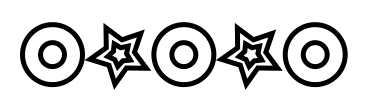Figure 23: A row constructed by alternating between two different images.

To do this we need to learn about a new method on `Int`. The modulo method, written `%`, returns the remainder of dividing one `Int` by another. Here are some examples.

``````4 % 2
// res1: Int = 0
3 % 2
// res2: Int = 1
2 % 2
// res3: Int = 0
1 % 2
// res4: Int = 1``````

We can see that when the first number is even the result is 0; otherwise it is 1. So we need to check is the result is 0 and act accordingly. There are a few ways to do this. Here’s one example

``````(4 % 2 == 0) match {
case true  => "It's even!"
case false => "It's odd!"
}
// res5: String = "It's even!"``````

Here we match against the result of the comparison `(4 % 2 == 0)`. The type of this expression is `Boolean`, which has two possible values (`true` and `false`).

For `Booleans` there is special syntax that is more compact than `match`: an `if` expression. Here’s the same code rewritten using `if`.

``````if(4 % 2 == 0) "It's even!"
else "It's odd!"
// res6: String = "It's even!"``````

Use whichever you are more comfortable with!

That’s all the background we need. Now we can write the method we’re interested in. Here’s the skeleton:

``````def alternatingRow(count: Int): Image =
???``````

Implement the method. It’s up to you what you choose for the two images used in the output.

Here’s my solution. I used an `if` expression because it’s a bit shorter than matching on the `Boolean`. Otherwise it’s the same structural recursion pattern as before.

``````val star = Image
.star(5, 30, 15, 45.degrees)
.strokeColor(Color.teal)
.on(Image.star(5, 12, 7, 45.degrees).strokeColor(Color.royalBlue))
.strokeWidth(5.0)

val circle = Image
.circle(60)
.strokeColor(Color.midnightBlue)
.on(Image.circle(24).strokeColor(Color.plum))
.strokeWidth(5.0)

def alternatingRow(count: Int): Image = {
count match {
case 0 => Image.empty
case n =>
if(n % 2 == 0) star.beside(alternatingRow(n-1))
else circle.beside(alternatingRow(n-1))
}
}``````

#### Getting Creative

We can use the counter to modify the image in other ways. For example we can make the color, size, or any othe property of an image depend on the counter. I have made an example in fig. 24, but come up with your own ideas. Implement a method

``````def funRow(count: Int): Image =
???``````

that generates such an image.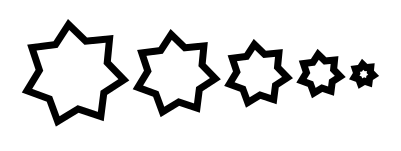Figure 24: A row constructed by making size and color depend on the counter.

Here’s my solution. I made the size of the star and its color depend on the counter.

``````def funRow(count: Int): Image = {
count match {
case 0 => Image.empty
case n =>
Image
.star(7, (10 * n), (7 * n), 45.degrees)
.strokeColor(Color.azure.spin((5 * n).degrees))
.strokeWidth(7.0)
.beside(funRow(n - 1))
}
}``````

#### Cross

Our final exercise is to create a method `cross` that will generate cross images. fig. 25 shows four cross images, which correspond to calling the method `cross` with `0` to `3`.

The method skeleton is

``````def cross(count: Int): Image =
???``````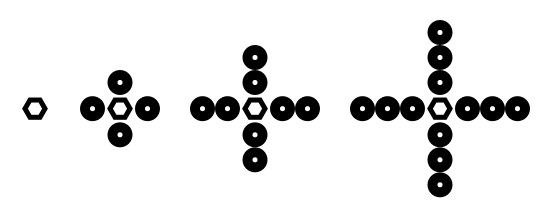Figure 25: Crosses generated by `count` from 0 to 3.

People often find this exercise harder than the preceding ones, so we’ll make the process very explicit here.

Firstly, what pattern will we use to fill in the body of `cross`? Write out the pattern.

It’s structural recursion over the natural numbers. You should end up with something like

``````def cross(count: Int): Image =
count match {
case 0 => <resultBase>
case n => <resultUnit> <add> cross(n-1)
}``````

Now we’ve identified the pattern we’re using, we need to fill in the problem specific parts:

• the base case; and
• the unit and addition operators.

Hint: use fig. 25 to identify the elements above.

From the picture we can work out that the base case is the hexagon in red.

Successive elements in the picture add circles to the top, bottom, left, and right of the image. So our unit is a circle, but the addition operator is not a simple `beside` or `above` like we’ve seen before but `unit.beside(unit.above(cross(n-1)).above(unit)).beside(unit)`.

Now finish the implementation of `cross`.

Here’s what we wrote.

``````def cross(count: Int): Image = {
count match {
case 0 =>
Image.regularPolygon(6, 10, 0.degrees)
.strokeColor(Color.deepSkyBlue.spin(-180.degrees))
.strokeWidth(5.0)
case n =>
val circle = Image
.circle(20)
.strokeColor(Color.deepSkyBlue)
.on(Image.circle(15).strokeColor(Color.deepSkyBlue.spin(-15.degrees)))
.on(Image.circle(10).strokeColor(Color.deepSkyBlue.spin(-30.degrees)))
.strokeWidth(5.0)
circle.beside(circle.above(cross(n - 1)).above(circle)).beside(circle)
}
}``````

## 7.5 Reasoning about Recursion

We’re now experienced users of structural recursion over the natural numbers. Let’s now return to our substitution model and see if it works with our new tool of recursion.

Recall that substitution says we can substitute the value of an expression wherever we see a value. In the case of a method call, we can substitute the body of the method with appropriate renaming of the parameters.

Our very first example of recursion was `boxes`, written like so:

``````val aBox = Image.square(20).fillColor(Color.royalBlue)

def boxes(count: Int): Image =
count match {
case 0 => Image.empty
case n => aBox.beside(boxes(n-1))
}``````

Let’s try using substitution on `boxes(3)` to see what we get.

Our first substitution is

``````boxes(3)
// Substitute body of `boxes`
3 match {
case 0 => Image.empty
case n => aBox.beside(boxes(n-1))
}``````

Knowing how to evaluate a `match` expression and using substitution again gives us

``````3 match {
case 0 => Image.empty
case n => aBox.beside(boxes(n-1))
}
// Substitute right-hand side expression of `case n`
aBox.beside(boxes(2))``````

We can substitute again on `boxes(2)` to obtain

``````aBox.beside(boxes(2))
// Substitute body of boxes
aBox.beside {
2 match {
case 0 => Image.empty
case n => aBox.beside(boxes(n-1))
}
}
// Substitute right-hand side expression of `case n`
aBox.beside {
aBox.beside(boxes(1))
}``````

Repeating the process a few more times we get

``````aBox.beside {
aBox.beside {
1 match {
case 0 => Image.empty
case n => aBox.beside(boxes(n-1))
}
}
}
// Substitute right-hand side expression of `case n`
aBox.beside {
aBox.beside {
aBox.beside(boxes(0))
}
}
// Substitute body of boxes
aBox.beside {
aBox.beside {
aBox.beside {
0 match {
case 0 => Image.empty
case n => aBox.beside(boxes(n-1))
}
}
}
}
// Substitute right-hand side expression of `case 0`
aBox.beside {
aBox.beside {
aBox.beside {
Image.empty
}
}
}``````

Our final result, which simplifies to

``aBox.beside(aBox).beside(aBox).beside(Image.empty)``

is exactly what we expect. Therefore we can say that substitution works to reason about recursion. This is great! However the substitutions are quite complex and difficult to keep track of without writing them down.

### 7.5.1 Reasoning About Structural Recursion

There is a more practical way to reason about structural recursion. Structural recursion guarantees the overall recursion is correct if we get the individual components correct. There are two parts to the structural recursion; the base case and the recursive case. The base case we can check just by looking at it. The recursive case has the recursive call (the method calling itself) but we don’t have to consider this. It is given to us by structural recursion so it will be correct so long as the other parts are correct. We can simply assume the recursive call it correct and then check that we are doing the right thing with the result of this call.

Let’s apply this to reasoning about `boxes`.

``````def boxes(count: Int): Image =
count match {
case 0 => Image.empty
case n => aBox.beside(boxes(n-1))
}``````

We can tell the base case is correct by inspection. Looking at the recursive case we assume that `boxes(n-1)` will do the right thing. The question then becomes: is what we do with the result of the recursive call `boxes(n-1)`, correct? The answer is yes: if the recursion `boxes(n-1)` creates `n-1` boxes in a line, sticking a box in front of them is the right thing to do. Since the individual cases are correct the whole thing is guaranted correct by structural recursion.

This way of reasoning is much more compact than using substitution and guaranteed to work if we’re using structural recursion.

### Exercises

Below are some rather silly examples of structural recursion. Work out if the methods do what they claim to do without running them.

``````// Given a natural number, returns that number
// Examples:
//   identity(0) == 0
//   identity(3) == 3
def identity(n: Int): Int =
n match {
case 0 => 0
case n => 1 + identity(n-1)
}``````

It sure does! The base case is straightforward. Looking at the recursive case, we assume that `identity(n-1)` returns the identity for `n-1` (which is exactly `n-1`). The identity for `n` is then `1 + identity(n-1)`.

``````// Given a natural number, double that number
// Examples:
//   double(0) == 0
//   double(3) == 6
def double(n: Int): Int =
n match {
case 0 => 0
case n => 2 * double(n-1)
}``````

No way! This method is broken in two different ways. Firstly, because we’re multiplying in the recursive case we will eventualy end up multiplying by base case of zero, and therefore the entire result will be zero.

We might try and fix this by adding a case for `1` (and perhaps wonder why the structural recursion skeleton let us down).

``````def double(n: Int): Int =
n match {
case 0 => 0
case 1 => 1
case n => 2 * double(n-1)
}``````

This doesn’t give us the correct result, however! We’re doing the wrong thing at the recursive case: we should be adding, not multiplying.

A bit of algebra:

``2(n-1 + 1) == 2(n-1) + 2``

So if `double(n-1)` is `2(n-1)` then we should add 2, not multiply by 2. The correct method is

``````def double(n: Int): Int =
n match {
case 0 => 0
case n => 2 + double(n-1)
}``````

## 7.6 Conclusions

In this chapter we’ve seen our first big pattern for structuring code, structural recursion over the natural numbers. There are a few key points.

First is the structural recursion pattern itself. We saw we can use this to write methods that produce a value with a varying size, and the pattern is the same everytime. We’ve seen a lot of examples that generate images, but we can use this pattern for anything that is transforming a natural number into anything else (including other natural numbers). We’ll use this pattern, and other variants of structural recursion, throughout the book.

The second big idea is how we reason about structural recursion. We can use substitution but it is easier to take a shortcut. For structural recursion we are guaranteed to get the correct result if we get the base case and the recursive case correct. In particular we don’t need to reason through the recursive call; we assume it returns the correct result and only check that we correctly implement the next step adding to the result.

The third key point is that we can use the value of the counter to do other things beyond the recursion. We looked at using it to adjust the images we created at each step, which gives us new creative possibilities.

In the next section we’ll look at creating more complex images using structural recursion, and see a few new techniques we can use with it.

# 8 Fractals

A fractal is an image that is self-similar, meaning that it contains copies of itself. Fractals are an intriguing type of image as they build complex output from simple rules. In this chapter we will build some simple fractals, get more experience with structural recursion over natural numbers, and finally learn more programming techniques.

If you run the examples from the SBT console within Doodle they will just work. If not, you will need to start your code with the following imports to make Doodle available.

``````import doodle.core._
import doodle.image._
import doodle.image.syntax._
import doodle.image.syntax.core._
import doodle.java2d._``````

## 8.1 The Chessboard

In this exercise and the next we’re trying to sharpen your eye for recursive structure.

Our first image is the chessboard. Though arguably not a fractal, the chessboard does contain itself: a 4x4 chessboard can be constructed from 4 2x2 chessboards, an 8x8 from 4 4x4s, and so on. The picture in fig. 26 shows this.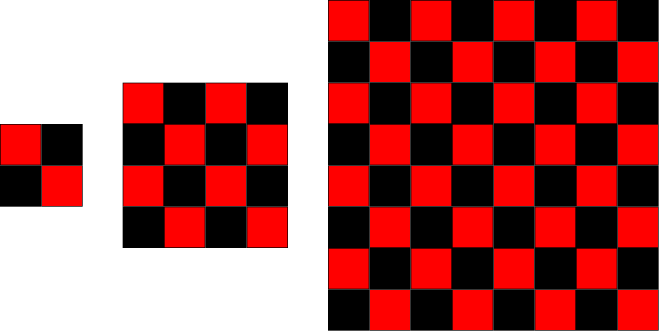Figure 26: Chessboards generated by `count` from 0 to 2.

Your mission in this exercise is to identify the recursive structure in a chessboard, and implement a method to draw chessboards. The method skeleton is

``````def chessboard(count: Int): Image =
???``````

Implement `chessboard`. Remember we can use the structural recursion skeleton and reasoning technique to guide our implementation.

`chessboard` is a structural recursion over the natural numbers, so right away we can write down the skeleton for this pattern.

``````def chessboard(count: Int): Image =
count match {
case 0 => resultBase
case n => resultUnit add chessboard(n-1)
}``````

As before we must decide on the base, unit, and addition for the result. We’ve given you a hint by showing the progression of chessboards in fig. ¿fig:recursion:chessboards?. From this we can see that the base is a two-by-two chessboard.

``````val blackSquare = Image.rectangle(30, 30).fillColor(Color.black)
val redSquare   = Image.rectangle(30, 30).fillColor(Color.red)

val base =
(redSquare.beside(blackSquare)).above(blackSquare.beside(redSquare))``````

Now to work out the unit and addition. Here we see a change from previous examples. The unit is the value we get from the recursive call `chessboard(n-1)`. The addition operation is `(unit beside unit) above (unit beside unit)`.

Putting it all together we get

``````def chessboard(count: Int): Image = {
val blackSquare = Image.rectangle(30, 30).fillColor(Color.black)
val redSquare   = Image.rectangle(30, 30).fillColor(Color.red)

val base =
(redSquare.beside(blackSquare)).above(blackSquare.beside(redSquare))
count match {
case 0 => base
case n =>
val unit = chessboard(n-1)
(unit.beside(unit)).above(unit.beside(unit))
}
}``````

If you have prior programming experience you might have immediately thought of creating a chessboard via two nested loops. Here we’re taking a different approach by defining a larger chessboard as a composition of smaller chessboards. Grasping this different approach to decomposing problems is a key step in becoming proficient in functional programming.

## 8.2 Sierpinkski Triangle

The Sierpinski triangle, show in fig. ¿fig:fractals:sierpinski?, is a famous fractal. (Technically, fig. ¿fig:fractals:sierpinski? shows a Sierpinkski triangle.)